# Chapter 3 Bar Graphs

Bar graphs are perhaps the most commonly used kind of data visualization. They’re typically used to display numeric values (on the y-axis), for different categories (on the x-axis). For example, a bar graph would be good for showing the prices of four different kinds of items. A bar graph generally wouldn’t be as good for showing prices over time, where time is a continuous variable – though it can be done, as we’ll see in this chapter.

There’s an important distinction you should be aware of when making bar graphs: sometimes the bar heights represent counts of cases in the data set, and sometimes they represent values in the data set. Keep this distinction in mind – it can be a source of confusion since they have very different relationships to the data, but the same term is used for both of them. In this chapter I’ll discuss this more, and present recipes for both types of bar graphs.

From this chapter on, this book will focus on using ggplot2 instead of base R graphics. Using ggplot2 will both keep things simpler and make for more sophisticated graphics.

## 3.1 Making a Basic Bar Graph

### 3.1.1 Problem

You have a data frame where one column represents the x position of each bar, and another column represents the vertical (y) height of each bar.

### 3.1.2 Solution

Use `ggplot()` with `geom_col()` and specify what variables you want on the x- and y-axes (Figure 3.1):

``````library(gcookbook)  # Load gcookbook for the pg_mean data set
ggplot(pg_mean, aes(x = group, y = weight)) +
geom_col()``````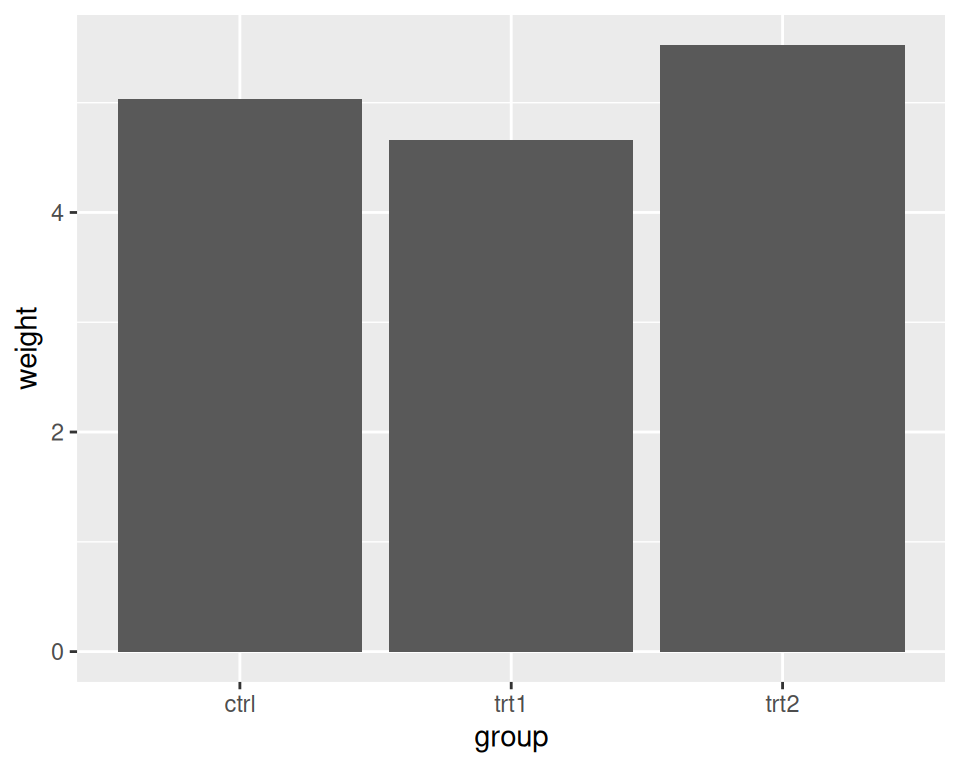Figure 3.1: Bar graph of values with a discrete x-axis

Note

In previous versions of ggplot2, the recommended way to create a bar graph of values was to use `geom_bar(stat = "identity")`. As of ggplot2 2.2.0, there is a `geom_col()` function which does the same thing.

### 3.1.3 Discussion

When x is a continuous (or numeric) variable, the bars behave a little differently. Instead of having one bar at each actual x value, there is one bar at each possible x value between the minimum and the maximum, as in Figure 3.2. You can convert the continuous variable to a discrete variable by using `factor()`.

``````# There's no entry for Time == 6
BOD
#>   Time demand
#> 1    1    8.3
#> 2    2   10.3
#> 3    3   19.0
#> 4    4   16.0
#> 5    5   15.6
#> 6    7   19.8

# Time is numeric (continuous)
str(BOD)
#> 'data.frame':    6 obs. of  2 variables:
#>  \$ Time  : num  1 2 3 4 5 7
#>  \$ demand: num  8.3 10.3 19 16 15.6 19.8
#>  - attr(*, "reference")= chr "A1.4, p. 270"

ggplot(BOD, aes(x = Time, y = demand)) +
geom_col()

# Convert Time to a discrete (categorical) variable with factor()
ggplot(BOD, aes(x = factor(Time), y = demand)) +
geom_col()``````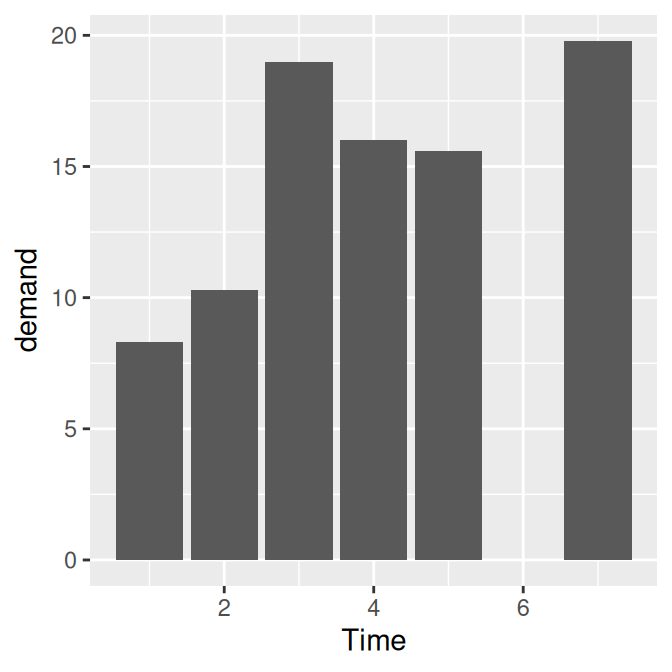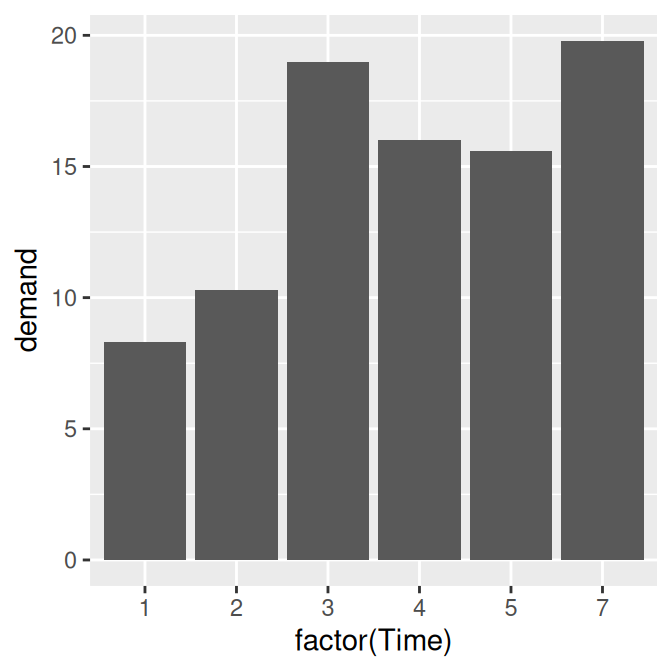Figure 3.2: Bar graph of values with a continuous x-axis (left); With x variable converted to a factor (notice that the space for 6 is gone; right)

Notice that there was no row in `BOD` for `Time` = 6. When the x variable is continuous, ggplot2 will use a numeric axis which will have space for all numeric values within the range – hence the empty space for 6 in the plot. When `Time` is converted to a factor, ggplot2 uses it as a discrete variable, where the values are treated as arbitrary labels instead of numeric values, and so it won’t allocate space on the x axis for all possible numeric values between the minimum and maximum.

In these examples, the data has a column for x values and another for y values. If you instead want the height of the bars to represent the count of cases in each group, see Recipe 3.3.

By default, bar graphs use a dark grey for the bars. To use a color fill, use `fill`. Also, by default, there is no outline around the fill. To add an outline, use `colour`. For Figure 3.3, we use a light blue fill and a black outline:

``````ggplot(pg_mean, aes(x = group, y = weight)) +
geom_col(fill = "lightblue", colour = "black")``````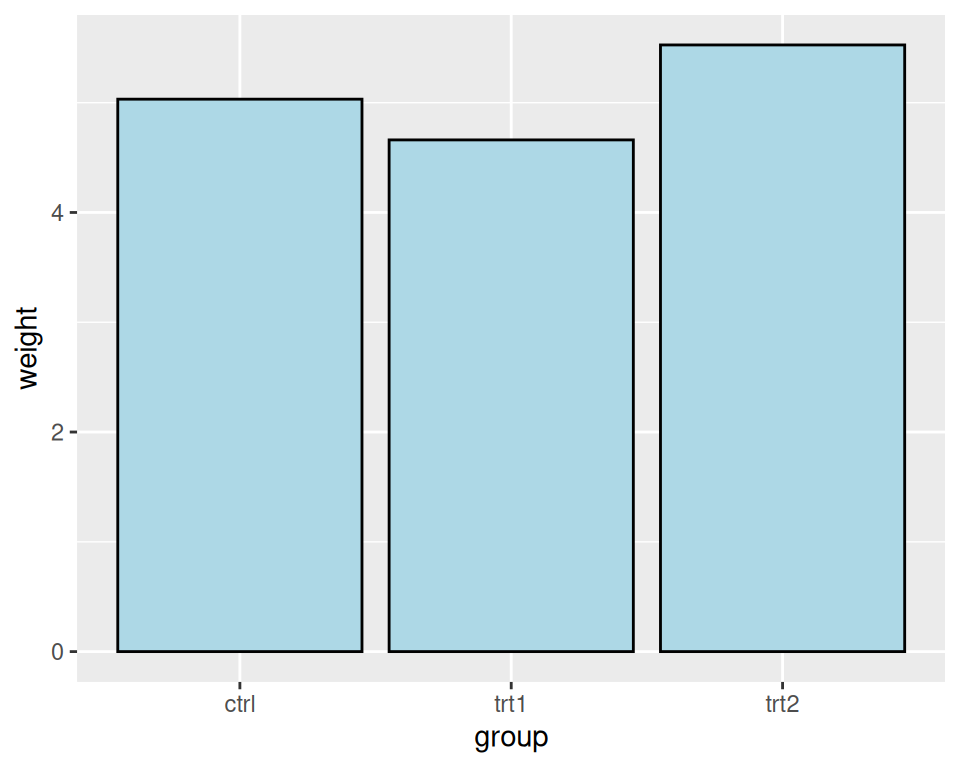Figure 3.3: A single fill and outline color for all bars

Note

In ggplot2, the default is to use the British spelling, colour, instead of the American spelling, color. Internally, American spellings are remapped to the British ones, so if you use the American spelling it will still work.

If you want the height of the bars to represent the count of cases in each group, see Recipe 3.3.

To reorder the levels of a factor based on the values of another variable, see Recipe 15.9. To manually change the order of factor levels, see Recipe 15.8.

## 3.2 Grouping Bars Together

### 3.2.1 Problem

You want to group bars together by a second variable.

### 3.2.2 Solution

Map a variable to fill, and use `geom_col(position = "dodge")`.

In this example we’ll use the `cabbage_exp` data set, which has two categorical variables, `Cultivar` and `Date`, and one continuous variable, `Weight`:

``````library(gcookbook)  # Load gcookbook for the cabbage_exp data set
cabbage_exp
#>   Cultivar Date Weight        sd  n         se
#> 1      c39  d16   3.18 0.9566144 10 0.30250803
#> 2      c39  d20   2.80 0.2788867 10 0.08819171
#> 3      c39  d21   2.74 0.9834181 10 0.31098410
#> 4      c52  d16   2.26 0.4452215 10 0.14079141
#> 5      c52  d20   3.11 0.7908505 10 0.25008887
#> 6      c52  d21   1.47 0.2110819 10 0.06674995``````

We’ll map `Date` to the x position and map `Cultivar` to the fill color (Figure 3.4):

``````ggplot(cabbage_exp, aes(x = Date, y = Weight, fill = Cultivar)) +
geom_col(position = "dodge")``````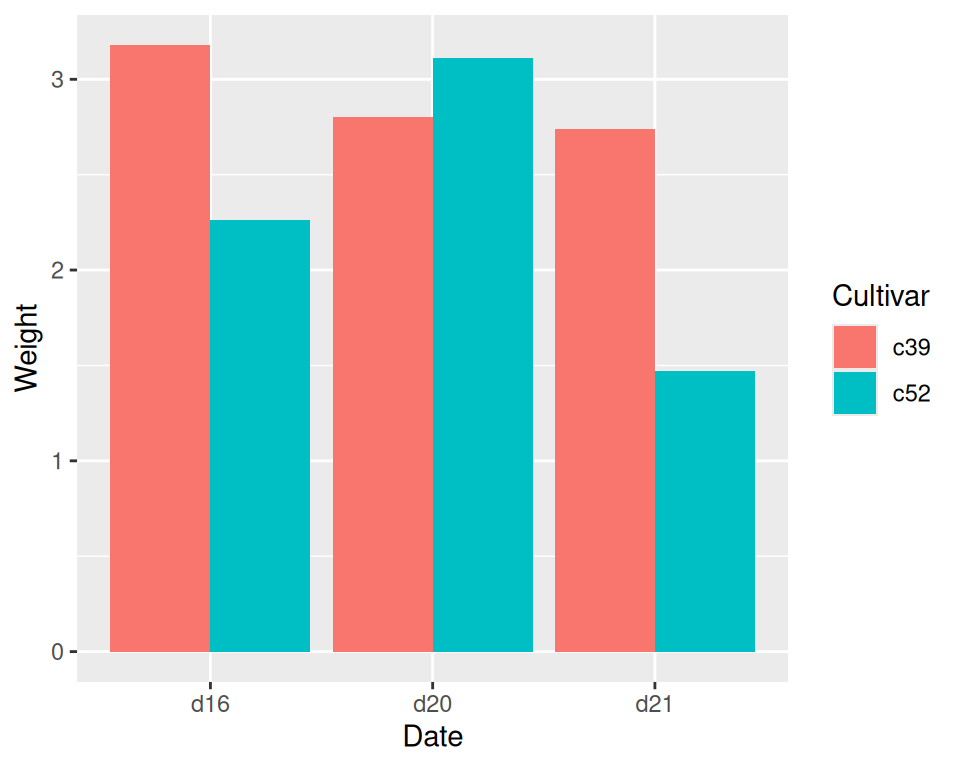Figure 3.4: Graph with grouped bars

### 3.2.3 Discussion

The most basic bar graphs have one categorical variable on the x-axis and one continuous variable on the y-axis. Sometimes you’ll want to use another categorical variable to divide up the data, in addition to the variable on the x-axis. You can produce a grouped bar plot by mapping that variable to fill, which represents the fill color of the bars. You must also use `position = "dodge"`, which tells the bars to “dodge” each other horizontally; if you don’t, you’ll end up with a stacked bar plot (Recipe 3.7).

As with variables mapped to the x-axis of a bar graph, variables that are mapped to the fill color of bars must be categorical rather than continuous variables.

To add a black outline, use `colour = "black"` inside `geom_col()`. To set the colors, you can use `scale_fill_brewer()` or `scale_fill_manual()`. In Figure 3.5 we’ll use the `Pastel1` palette from `RColorBrewer`:

``````ggplot(cabbage_exp, aes(x = Date, y = Weight, fill = Cultivar)) +
geom_col(position = "dodge", colour = "black") +
scale_fill_brewer(palette = "Pastel1")``````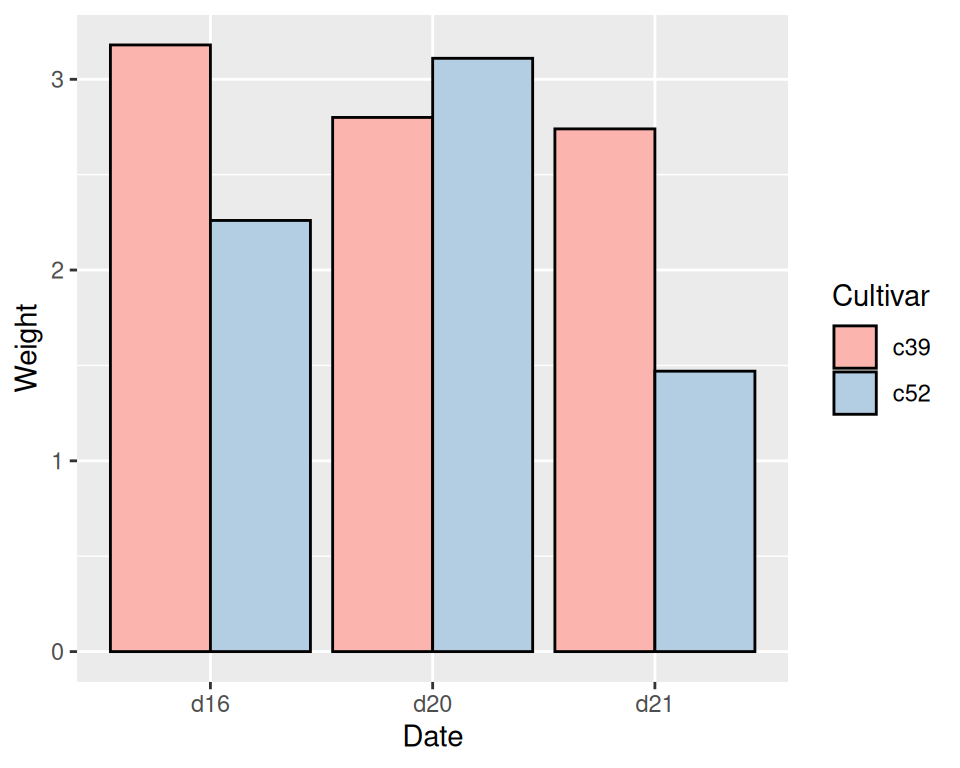Figure 3.5: Grouped bars with black outline and a different color palette

Other aesthetics, such as `colour` (the color of the outlines of the bars) or `linestyle`, can also be used for grouping variables, but `fill` is probably what you’ll want to use.

Note that if there are any missing combinations of the categorical variables, that bar will be missing, and the neighboring bars will expand to fill that space. If we remove the last row from our example data frame, we get Figure 3.6:

``````ce <- cabbage_exp[1:5, ]
ce
#>   Cultivar Date Weight        sd  n         se
#> 1      c39  d16   3.18 0.9566144 10 0.30250803
#> 2      c39  d20   2.80 0.2788867 10 0.08819171
#> 3      c39  d21   2.74 0.9834181 10 0.31098410
#> 4      c52  d16   2.26 0.4452215 10 0.14079141
#> 5      c52  d20   3.11 0.7908505 10 0.25008887

ggplot(ce, aes(x = Date, y = Weight, fill = Cultivar)) +
geom_col(position = "dodge", colour = "black") +
scale_fill_brewer(palette = "Pastel1")``````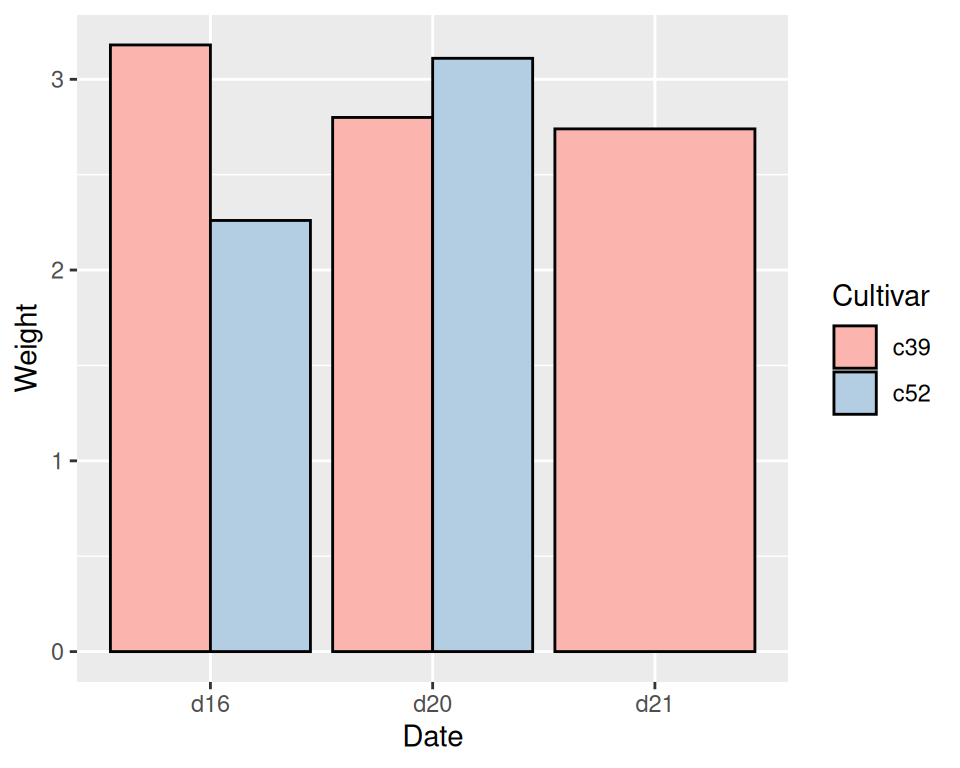Figure 3.6: Graph with a missing bar-the other bar fills the space

If your data has this issue, you can manually make an entry for the missing factor level combination with an `NA` for the y variable.

For more on using colors in bar graphs, see Recipe 3.4.

To reorder the levels of a factor based on the values of another variable, see Recipe 15.9.

## 3.3 Making a Bar Graph of Counts

### 3.3.1 Problem

Your data has one row representing each case, and you want plot counts of the cases.

### 3.3.2 Solution

Use `geom_bar()` without mapping anything to `y` (Figure 3.7):

``````# Equivalent to using geom_bar(stat = "bin")
ggplot(diamonds, aes(x = cut)) +
geom_bar()``````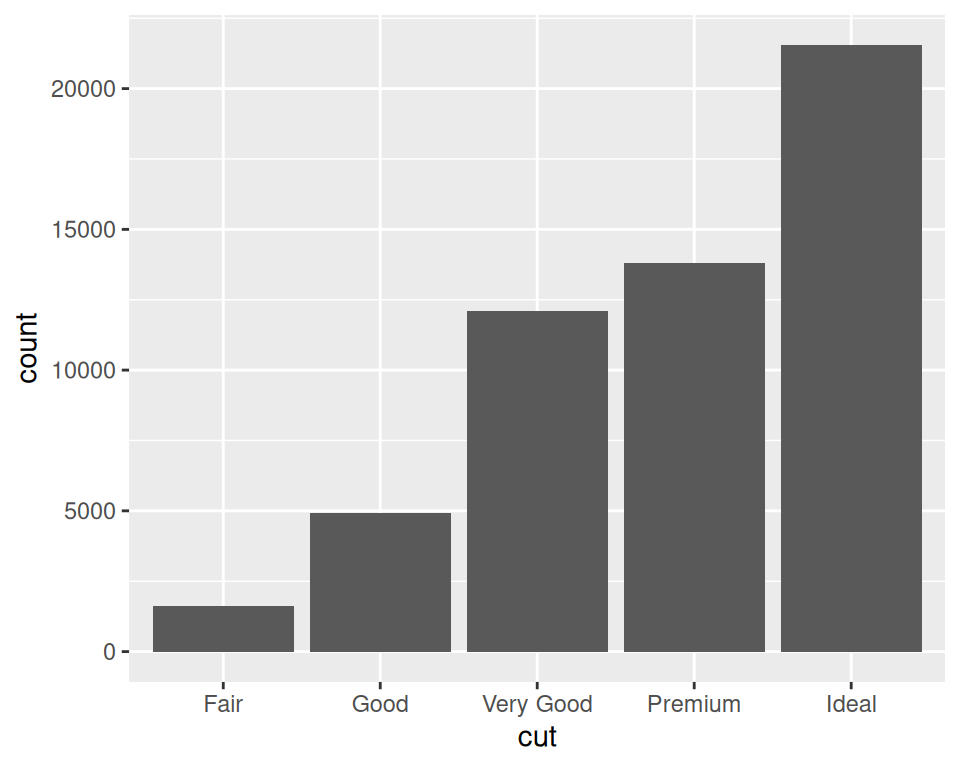Figure 3.7: Bar graph of counts

### 3.3.3 Discussion

The `diamonds` data set has 53,940 rows, each of which represents information about a single diamond:

``````diamonds
#> # A tibble: 53,940 x 10
#>   carat cut       color clarity depth table price     x     y     z
#>   <dbl> <ord>     <ord> <ord>   <dbl> <dbl> <int> <dbl> <dbl> <dbl>
#> 1 0.23  Ideal     E     SI2      61.5    55   326  3.95  3.98  2.43
#> 2 0.21  Premium   E     SI1      59.8    61   326  3.89  3.84  2.31
#> 3 0.23  Good      E     VS1      56.9    65   327  4.05  4.07  2.31
#> 4 0.290 Premium   I     VS2      62.4    58   334  4.2   4.23  2.63
#> 5 0.31  Good      J     SI2      63.3    58   335  4.34  4.35  2.75
#> 6 0.24  Very Good J     VVS2     62.8    57   336  3.94  3.96  2.48
#> # … with 5.393e+04 more rows``````

With `geom_bar()`, the default behavior is to use `stat = "bin"`, which counts up the number of cases for each group (each x position, in this example). In the graph we can see that there are about 23,000 cases with an `ideal` cut.

In this example, the variable on the x-axis is discrete. If we use a continuous variable on the x-axis, we’ll get a bar at each unique x value in the data, as shown in Figure 3.8, left: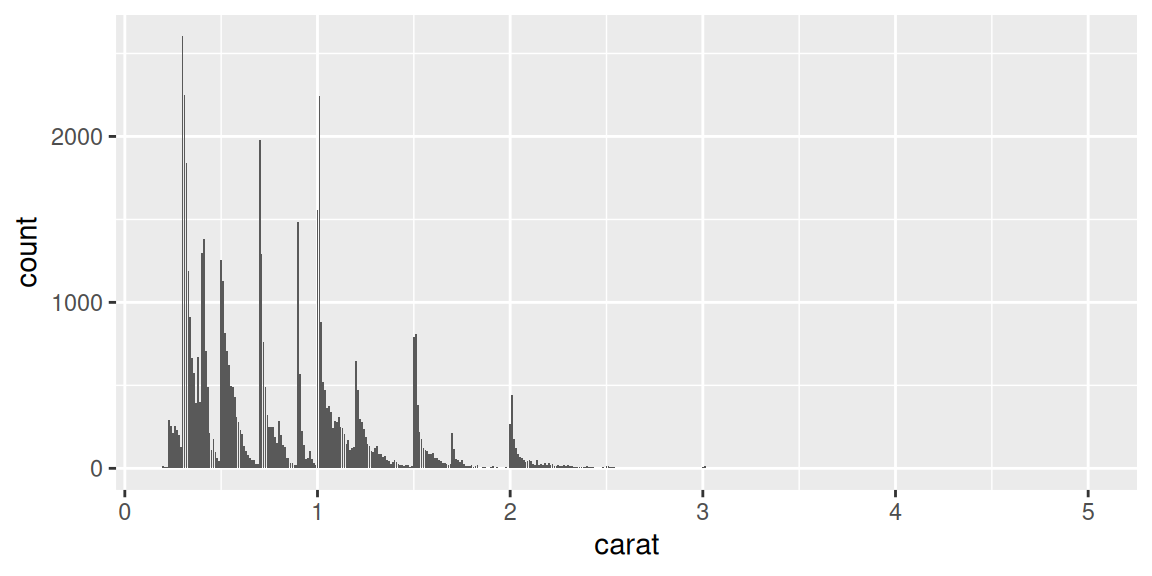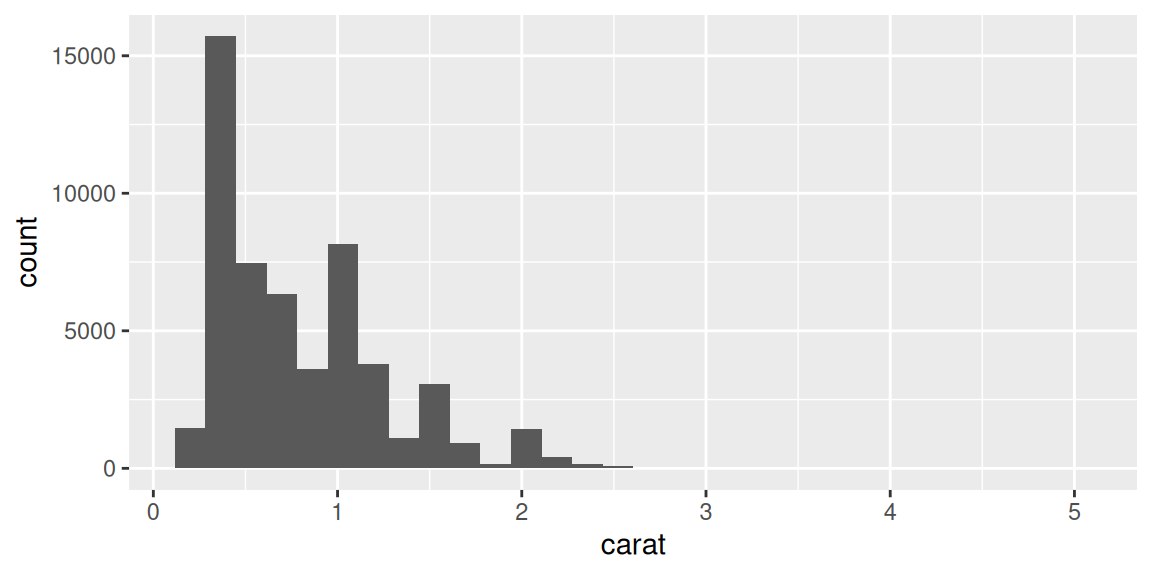Figure 3.8: Bar graph of counts on a continuous axis (left); A histogram (right)

The bar graph with a continuous x-axis is similar to a histogram, but not the same. A histogram is shown on the right of Figure 3.8. In this kind of bar graph, each bar represents a unique x value, whereas in a histogram, each bar represents a range of x values.

If, instead of having `ggplot()` count up the number of rows in each group, you have a column in your data frame representing the y values, use `geom_col()`. See Recipe 3.1.

You could also get the same graphical output by calculating the counts before sending the data to `ggplot()`. See Recipe 15.17 for more on summarizing data.

For more about histograms, see Recipe 6.1.

## 3.4 Using Colors in a Bar Graph

### 3.4.1 Problem

You want to use different colors for the bars in your graph.

### 3.4.2 Solution

Map the appropriate variable to the fill aesthetic.

We’ll use the `uspopchange` data set for this example. It contains the percentage change in population for the US states from 2000 to 2010. We’ll take the top 10 fastest-growing states and graph their percentage change. We’ll also color the bars by region (Northeast, South, North Central, or West).

First, take the top 10 states:

``````library(gcookbook) # Load gcookbook for the uspopchange data set
library(dplyr)

upc <- uspopchange %>%
arrange(desc(Change)) %>%
slice(1:10)

upc
#>             State Abb Region Change
#> 1          Nevada  NV   West   35.1
#> 2         Arizona  AZ   West   24.6
#> 3            Utah  UT   West   23.8
#>  ...<4 more rows>...
#> 8         Florida  FL  South   17.6
#> 9        Colorado  CO   West   16.9
#> 10 South Carolina  SC  South   15.3``````

Now we can make the graph, mapping Region to fill (Figure 3.9):

``````ggplot(upc, aes(x = Abb, y = Change, fill = Region)) +
geom_col()``````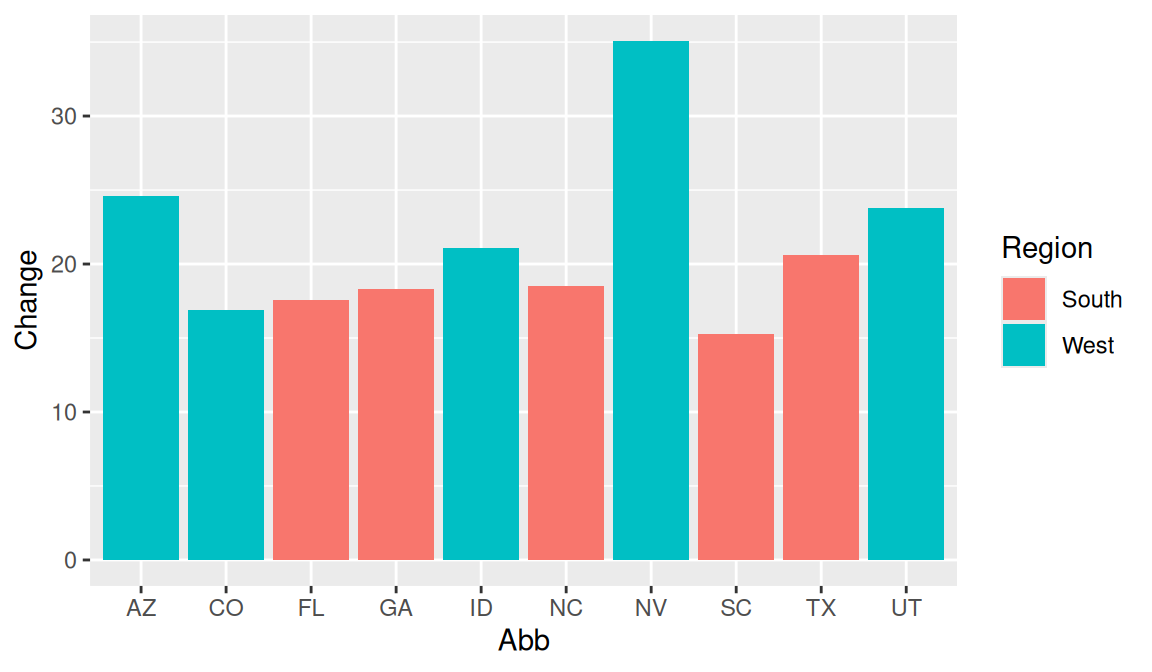Figure 3.9: A variable mapped to fill

### 3.4.3 Discussion

The default colors aren’t the most appealing, so you may want to set them using `scale_fill_brewer()` or `scale_fill_manual()`. With this example, we’ll use the latter, and we’ll set the outline color of the bars to black, with `colour="black"` (Figure 3.10). Note that setting occurs outside of `aes()`, while mapping occurs within `aes()`:

``````ggplot(upc, aes(x = reorder(Abb, Change), y = Change, fill = Region)) +
geom_col(colour = "black") +
scale_fill_manual(values = c("#669933", "#FFCC66")) +
xlab("State")``````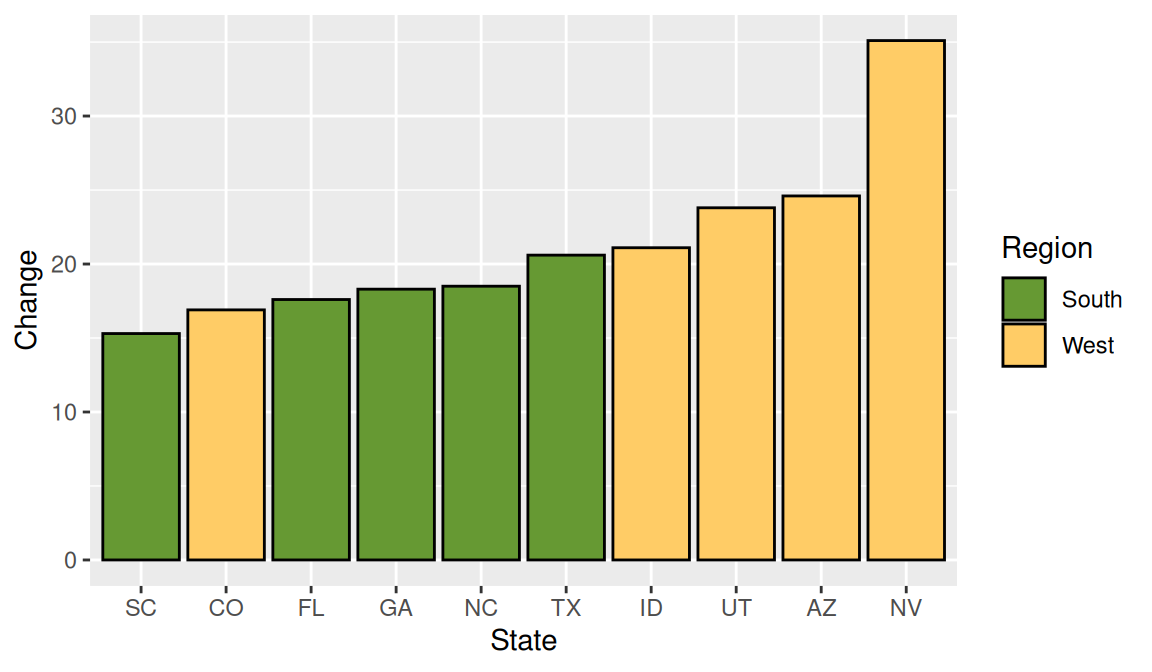Figure 3.10: Graph with different colors, black outlines, and sorted by percentage change

This example also uses the `reorder()` function to reorder the levels of the factor `Abb` based on the values of `Change`. In this particular case it makes sense to sort the bars by their height, instead of in alphabetical order.

For more about using `reorder()`, see Recipe 15.9.

## 3.5 Coloring Negative and Positive Bars Differently

### 3.5.1 Problem

You want to use different colors for negative and positive-valued bars.

### 3.5.2 Solution

We’ll use a subset of the climate data and create a new column called pos, which indicates whether the value is positive or negative:

``````library(gcookbook) # Load gcookbook for the climate data set
library(dplyr)

climate_sub <- climate %>%
filter(Source == "Berkeley" & Year >= 1900) %>%
mutate(pos = Anomaly10y >= 0)

climate_sub
#>       Source Year Anomaly1y Anomaly5y Anomaly10y Unc10y   pos
#> 1   Berkeley 1900        NA        NA     -0.171  0.108 FALSE
#> 2   Berkeley 1901        NA        NA     -0.162  0.109 FALSE
#> 3   Berkeley 1902        NA        NA     -0.177  0.108 FALSE
#>  ...<99 more rows>...
#> 103 Berkeley 2002        NA        NA      0.856  0.028  TRUE
#> 104 Berkeley 2003        NA        NA      0.869  0.028  TRUE
#> 105 Berkeley 2004        NA        NA      0.884  0.029  TRUE``````

Once we have the data, we can make the graph and map pos to the fill color, as in Figure 3.11. Notice that we use position=“identity” with the bars. This will prevent a warning message about stacking not being well defined for negative numbers:

``````ggplot(climate_sub, aes(x = Year, y = Anomaly10y, fill = pos)) +
geom_col(position = "identity")``````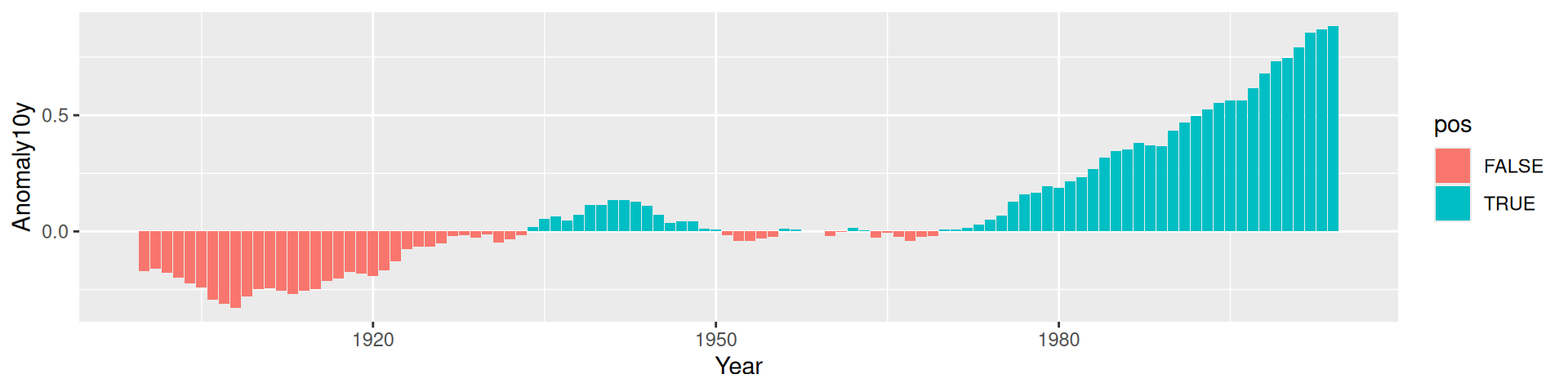Figure 3.11: Different colors for positive and negative values

### 3.5.3 Discussion

There are a few problems with the first attempt. First, the colors are probably the reverse of what we want: usually, blue means cold and red means hot. Second, the legend is redundant and distracting.

We can change the colors with `scale_fill_manual()` and remove the legend with `guide = FALSE`, as shown in Figure 3.12. We’ll also add a thin black outline around each of the bars by setting `colour` and specifying `size`, which is the thickness of the outline (in millimeters):

``````ggplot(climate_sub, aes(x = Year, y = Anomaly10y, fill = pos)) +
geom_col(position = "identity", colour = "black", size = 0.25) +
scale_fill_manual(values = c("#CCEEFF", "#FFDDDD"), guide = FALSE)``````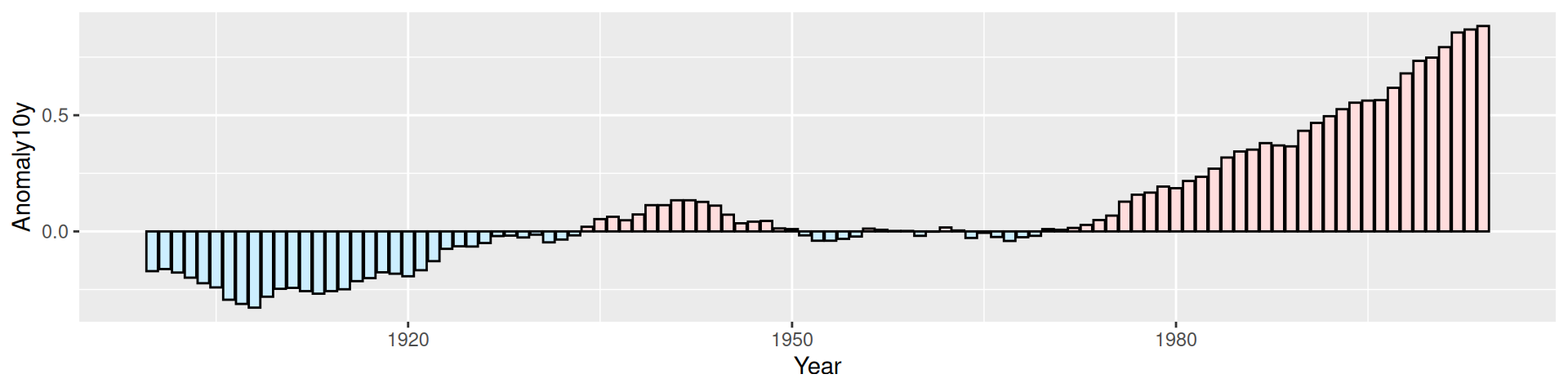Figure 3.12: Graph with customized colors and no legend

To change the colors used, see Recipes Recipe 12.4 and Recipe 12.5.

To hide the legend, see Recipe 10.1.

## 3.6 Adjusting Bar Width and Spacing

### 3.6.1 Problem

You want to adjust the width of bars and the spacing between them.

### 3.6.2 Solution

To make the bars narrower or wider, set `width` in `geom_bar()`. The default value is 0.9; larger values make the bars wider, and smaller values make the bars narrower (Figure 3.13).

For example, for standard-width bars:

``````library(gcookbook) # Load gcookbook for the pg_mean data set

ggplot(pg_mean, aes(x = group, y = weight)) +
geom_col()``````

For narrower bars:

``````ggplot(pg_mean, aes(x = group, y = weight)) +
geom_col(width = 0.5)``````

And for wider bars (these have the maximum width of 1):

``````ggplot(pg_mean, aes(x = group, y = weight)) +
geom_col(width = 1)``````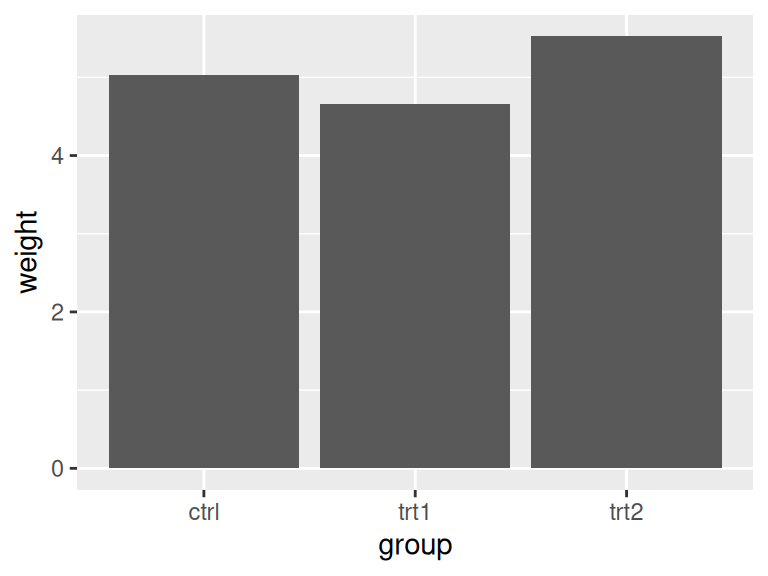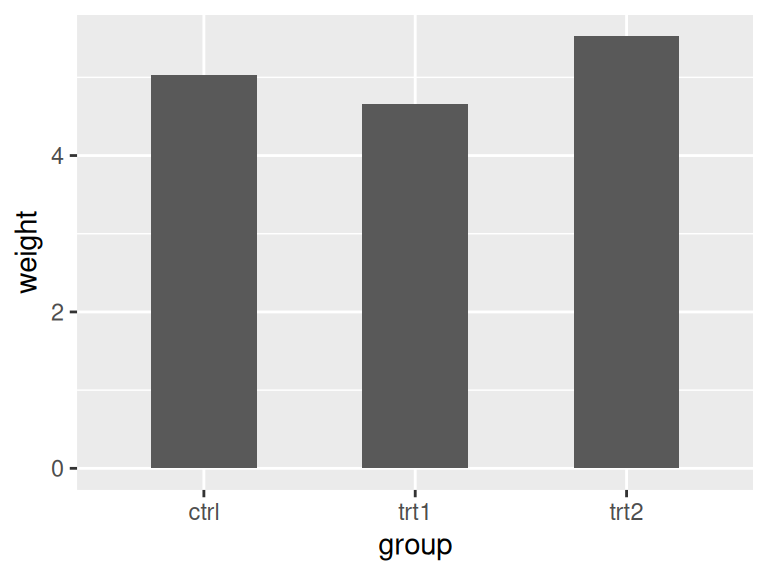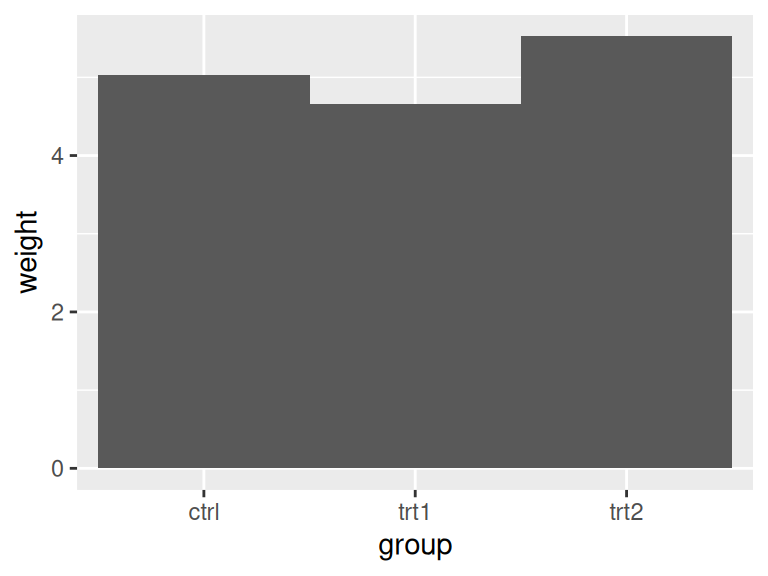Figure 3.13: Different bar widths

For grouped bars, the default is to have no space between bars within each group. To add space between bars within a group, make width smaller and set the value for `position_dodge` to be larger than `width` (Figure 3.14).

For a grouped bar graph with narrow bars:

``````ggplot(cabbage_exp, aes(x = Date, y = Weight, fill = Cultivar)) +
geom_col(width = 0.5, position = "dodge")``````

And with some space between the bars:

``````ggplot(cabbage_exp, aes(x = Date, y = Weight, fill = Cultivar)) +
geom_col(width = 0.5, position = position_dodge(0.7))``````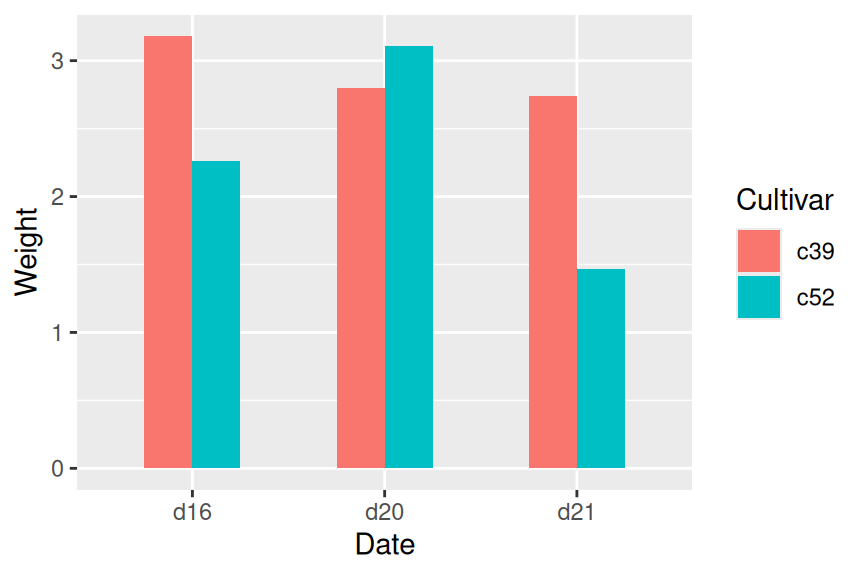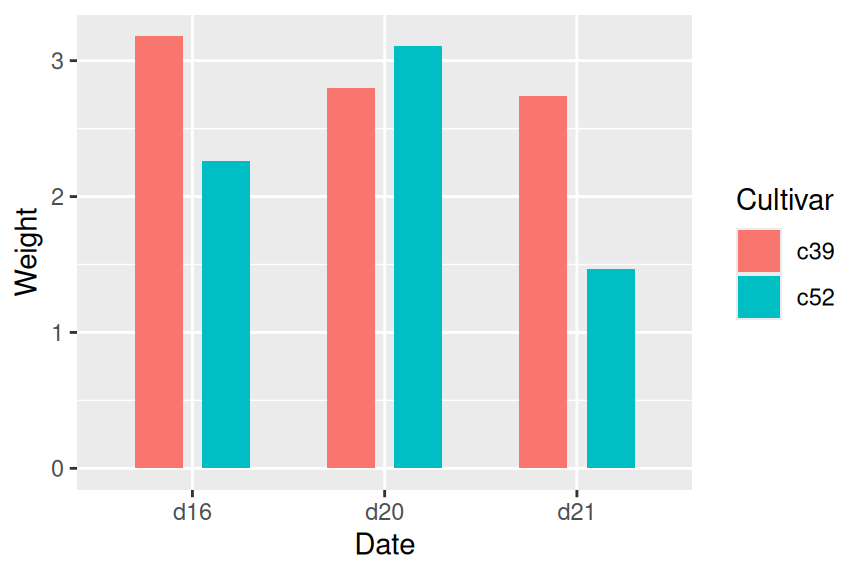Figure 3.14: Bar graph with narrow grouped bars (left); With space between the bars (right)

The first graph used `position = "dodge"`, and the second graph used `position = position_dodge()`. This is because `position = "dodge"` is simply shorthand for `position = position_dodge()` with the default value of 0.9, but when we want to set a specific value, we need to use the more verbose form.

### 3.6.3 Discussion

The default `width` for bars is 0.9, and the default value used for `position_dodge()` is the same. To be more precise, the value of `width` in `position_dodge()` is `NULL`, which tells ggplot2 to use the same value as the width from `geom_bar()`.

All of these will have the same result:

``````geom_bar(position = "dodge")
geom_bar(width = 0.9, position = position_dodge())
geom_bar(position = position_dodge(0.9))
geom_bar(width = 0.9, position = position_dodge(width=0.9))``````

The items on the x-axis have x values of 1, 2, 3, and so on, though you typically don’t refer to them by these numerical values. When you use `geom_bar(width = 0.9)`, it makes each group take up a total width of 0.9 on the x-axis. When you use `position_dodge(width = 0.9)`, it spaces the bars so that the middle of each bar is right where it would be if the bar width were 0.9 and the bars were touching. This is illustrated in Figure 3.15. The two graphs both have the same dodge width of 0.9, but while the top has a bar width of 0.9, the bottom has a bar width of 0.2. Despite the different bar widths, the middles of the bars stay aligned.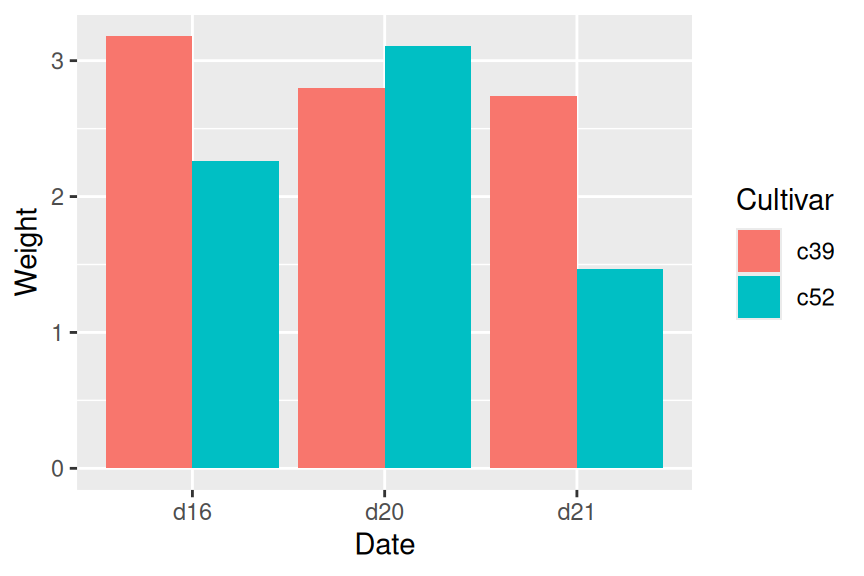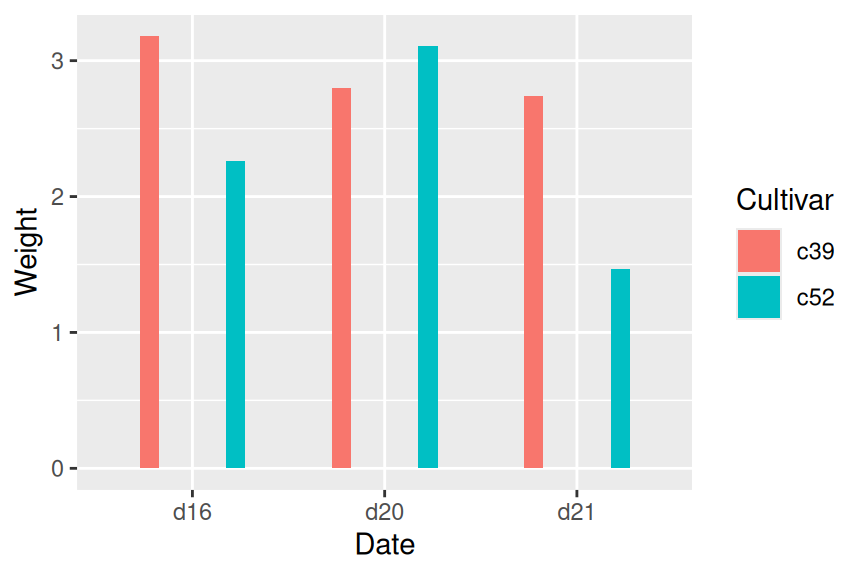Figure 3.15: Same dodge width of 0.9, but different bar widths of 0.9 (top) and 0.2 (bottom)

If you make the entire graph wider or narrower, the bar dimensions will scale proportionally. To see how this works, you can just resize the window in which the graphs appear. For information about controlling this when writing to a file, see Chapter 14.

## 3.7 Making a Stacked Bar Graph

### 3.7.1 Problem

You want to make a stacked bar graph.

### 3.7.2 Solution

Use `geom_bar()` and map a variable `fill`. This will put `Date` on the x-axis and use `Cultivar` for the fill color, as shown in Figure 3.16:

``````library(gcookbook) # Load gcookbook for the cabbage_exp data set

ggplot(cabbage_exp, aes(x = Date, y = Weight, fill = Cultivar)) +
geom_col()``````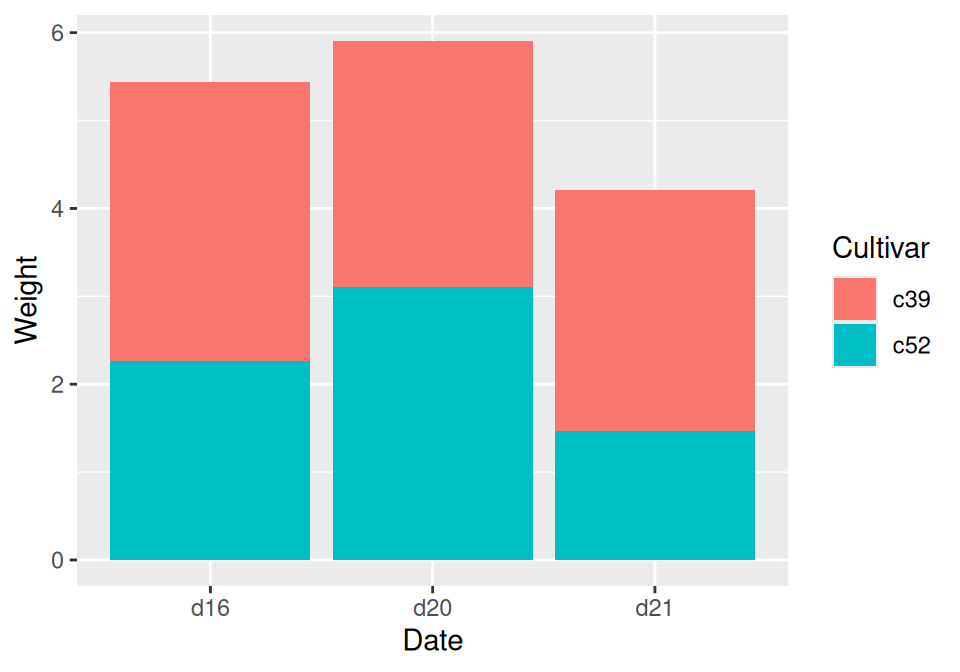Figure 3.16: Stacked bar graph

### 3.7.3 Discussion

To understand how the graph is made, it’s useful to see how the data is structured. There are three levels of `Date` and two levels of `Cultivar`, and for each combination there is a value for `Weight`:

``````cabbage_exp
#>   Cultivar Date Weight        sd  n         se
#> 1      c39  d16   3.18 0.9566144 10 0.30250803
#> 2      c39  d20   2.80 0.2788867 10 0.08819171
#> 3      c39  d21   2.74 0.9834181 10 0.31098410
#> 4      c52  d16   2.26 0.4452215 10 0.14079141
#> 5      c52  d20   3.11 0.7908505 10 0.25008887
#> 6      c52  d21   1.47 0.2110819 10 0.06674995``````

By default, the stacking order of the bars is the same as the order of items in the legend. For some data sets it might make sense to reverse the order of the legend. To do this, you can use the `guides` function and specify which aesthetic for which the legend should be reversed. In this case, it’s `fill`:

``````ggplot(cabbage_exp, aes(x = Date, y = Weight, fill = Cultivar)) +
geom_col() +
guides(fill = guide_legend(reverse = TRUE))``````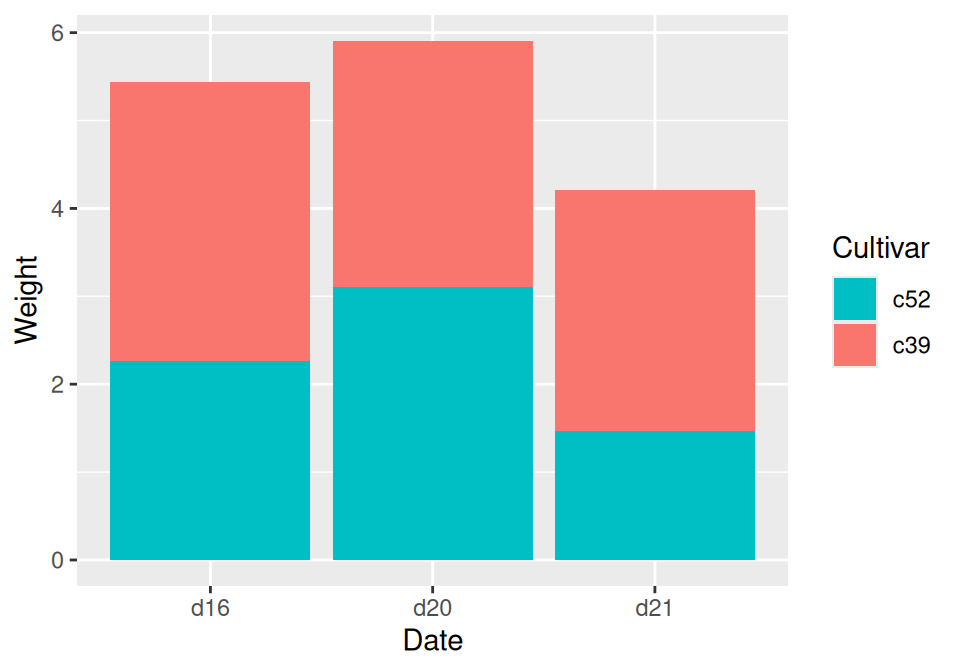Figure 3.17: Stacked bar graph with reversed legend order

If you’d like to reverse the stacking order of the bars, as in Figure 3.18, use `position_stack(reverse = TRUE)`. You’ll also need to reverse the order of the legend for it to match the order of the bars:

``````ggplot(cabbage_exp, aes(x = Date, y = Weight, fill = Cultivar)) +
geom_col(position = position_stack(reverse = TRUE)) +
guides(fill = guide_legend(reverse = TRUE))``````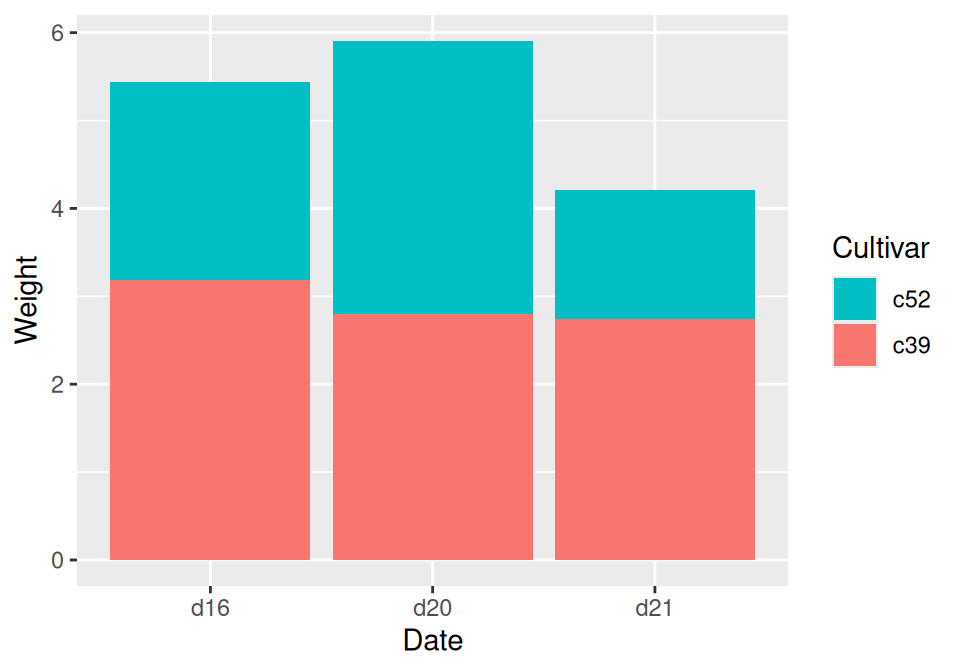Figure 3.18: Stacked bar graph with reversed stacking order

It’s also possible to modify the column of the data frame so that the factor levels are in a different order (see Recipe 15.8). Do this with care, since the modified data could change the results of other analyses.

For a more polished graph, we’ll use `scale_fill_brewer()` to get a different color palette, and use `colour="black"` to get a black outline (Figure 3.19):

``````ggplot(cabbage_exp, aes(x = Date, y = Weight, fill = Cultivar)) +
geom_col(colour = "black") +
scale_fill_brewer(palette = "Pastel1")``````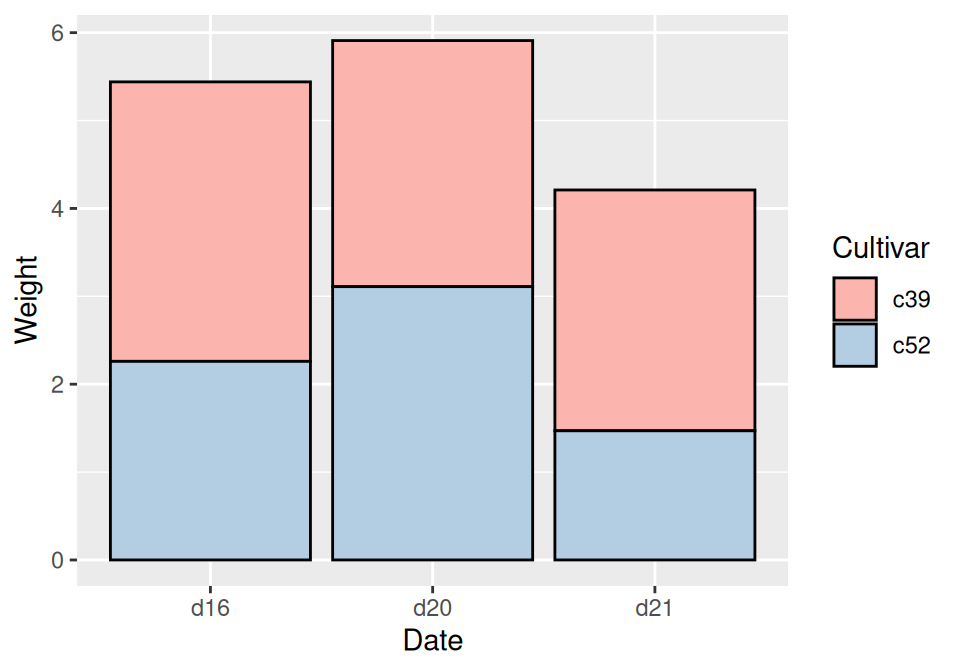Figure 3.19: Stacked bar graph with reversed legend, new palette, and black outline

For more on using colors in bar graphs, see Recipe 3.4.

To reorder the levels of a factor based on the values of another variable, see Recipe 15.9. To manually change the order of factor levels, see Recipe 15.8.

## 3.8 Making a Proportional Stacked Bar Graph

### 3.8.1 Problem

You want to make a stacked bar graph that shows proportions (also called a 100% stacked bar graph).

### 3.8.2 Solution

Use `geom_col(position = "fill")` (Figure 3.20):

``````library(gcookbook) # Load gcookbook for the cabbage_exp data set

ggplot(cabbage_exp, aes(x = Date, y = Weight, fill = Cultivar)) +
geom_col(position = "fill")``````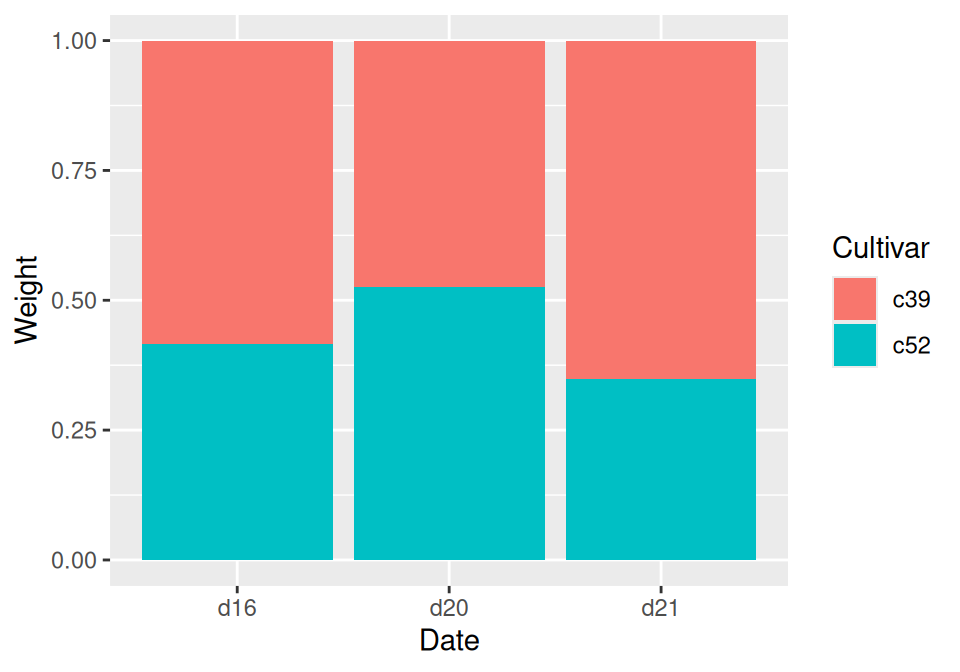Figure 3.20: Proportional stacked bar graph

### 3.8.3 Discussion

With `position = "fill"`, the y values will be scaled to go from 0 to 1. To print the labels as percentages, use `scale_y_continuous(labels = scales::percent)`.

``````ggplot(cabbage_exp, aes(x = Date, y = Weight, fill = Cultivar)) +
geom_col(position = "fill") +
scale_y_continuous(labels = scales::percent)``````

Note

Using `scales::percent` is a way of using the `percent` function from the scales package. You could instead do `library(scales)` and then just use `scale_y_continuous(labels = percent)`. This would also make all of the functions from scales available in the current R session.

To make the output look a little nicer, you can change the color palette and add an outline. This is shown in (Figure 3.21):

``````ggplot(cabbage_exp, aes(x = Date, y = Weight, fill = Cultivar)) +
geom_col(colour = "black", position = "fill") +
scale_y_continuous(labels = scales::percent) +
scale_fill_brewer(palette = "Pastel1")``````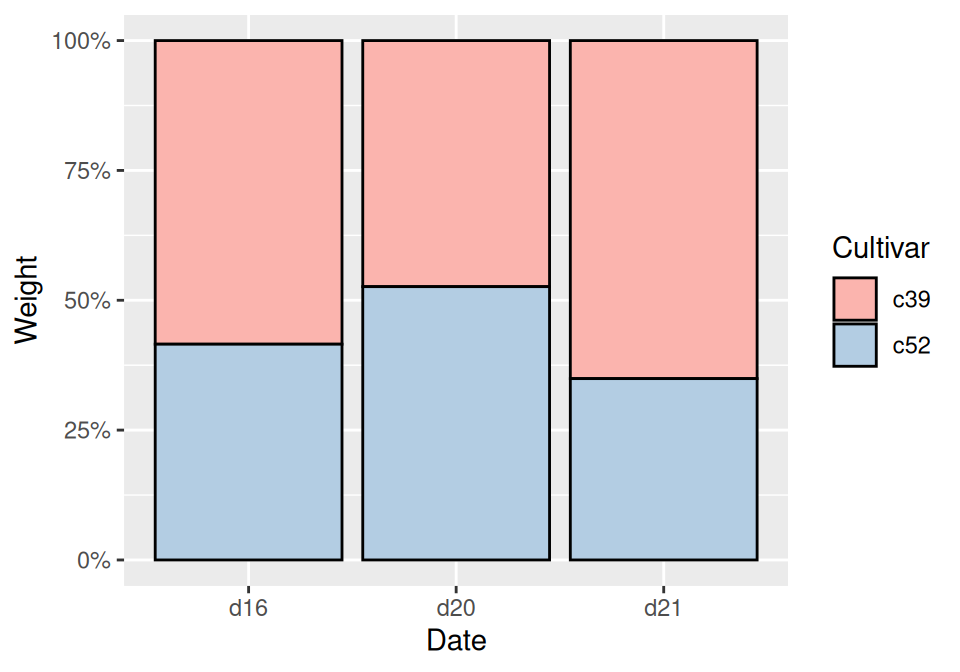Figure 3.21: Proportional stacked bar graph with reversed legend, new palette, and black outline

Instead of having ggplot2 compute the proportions automatically, you may want to compute the proportional values yourself. This can be useful if you want to use those values in other computations.

To do this, first scale the data to 100% within each stack. This can be done by using `group_by()` together with `mutate()` from the dplyr package.

``````library(gcookbook)
library(dplyr)

cabbage_exp
#>   Cultivar Date Weight        sd  n         se
#> 1      c39  d16   3.18 0.9566144 10 0.30250803
#> 2      c39  d20   2.80 0.2788867 10 0.08819171
#> 3      c39  d21   2.74 0.9834181 10 0.31098410
#> 4      c52  d16   2.26 0.4452215 10 0.14079141
#> 5      c52  d20   3.11 0.7908505 10 0.25008887
#> 6      c52  d21   1.47 0.2110819 10 0.06674995

# Do a group-wise transform(), splitting on "Date"
ce <- cabbage_exp %>%
group_by(Date) %>%
mutate(percent_weight = Weight / sum(Weight) * 100)

ce
#> # A tibble: 6 x 7
#> # Groups:   Date 
#>   Cultivar Date  Weight    sd     n     se percent_weight
#>   <fct>    <fct>  <dbl> <dbl> <int>  <dbl>          <dbl>
#> 1 c39      d16     3.18 0.957    10 0.303            58.5
#> 2 c39      d20     2.8  0.279    10 0.0882           47.4
#> 3 c39      d21     2.74 0.983    10 0.311            65.1
#> 4 c52      d16     2.26 0.445    10 0.141            41.5
#> 5 c52      d20     3.11 0.791    10 0.250            52.6
#> 6 c52      d21     1.47 0.211    10 0.0667           34.9``````

To calculate the percentages within each `Weight` group, we used dplyr’s `group_by()` and `mutate()` functions. In the example here, the `group_by()` function tells dplyr that future operations should operate on the data frame as though it were split up into groups, on the `Date` column. The `mutate()` function tells it to calculate a new column, dividing each row’s `Weight` value by the sum of the `Weight` column within each group.

Note

You may have noticed that `cabbage_exp` and `ce` print out differently. This is because `cabbage_exp` is a regular data frame, while `ce` is a tibble, which is a data frame with some extra properties. The dplyr package creates tibbles. For more information, see Chapter 15.

After computing the new column, making the graph is the same as with a regular stacked bar graph.

``````ggplot(ce, aes(x = Date, y = percent_weight, fill = Cultivar)) +
geom_col()``````

For more on transforming data by groups, see Recipe 15.16.

## 3.9 Adding Labels to a Bar Graph

### 3.9.1 Problem

You want to add labels to the bars in a bar graph.

### 3.9.2 Solution

Add `geom_text()` to your graph. It requires a mapping for x, y, and the text itself. By setting `vjust` (the vertical justification), it is possible to move the text above or below the tops of the bars, as shown in Figure 3.22:

``````library(gcookbook) # Load gcookbook for the cabbage_exp data set

# Below the top
ggplot(cabbage_exp, aes(x = interaction(Date, Cultivar), y = Weight)) +
geom_col() +
geom_text(aes(label = Weight), vjust = 1.5, colour = "white")

# Above the top
ggplot(cabbage_exp, aes(x = interaction(Date, Cultivar), y = Weight)) +
geom_col() +
geom_text(aes(label = Weight), vjust = -0.2)``````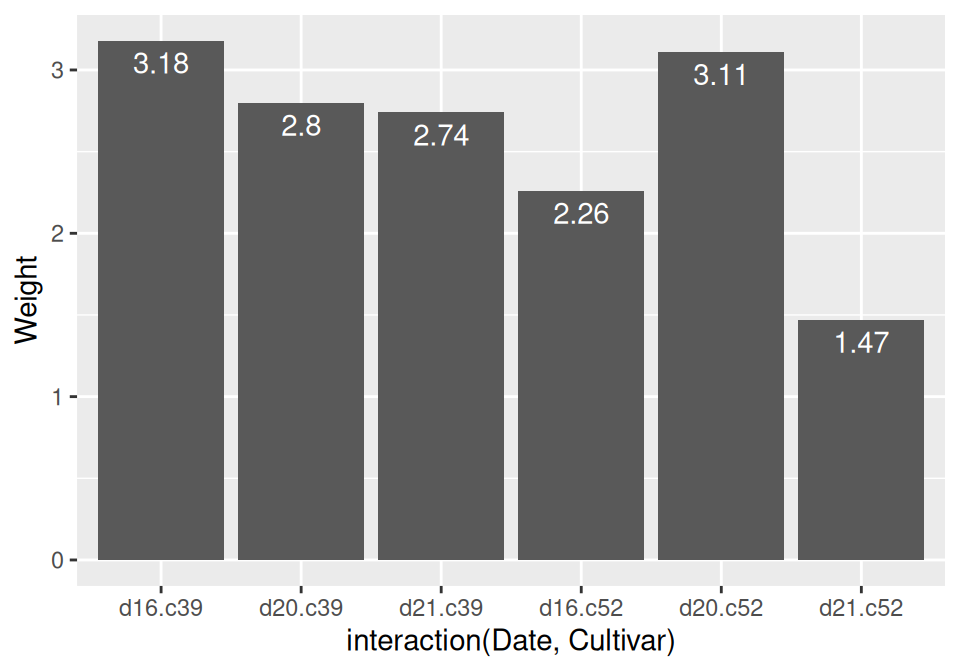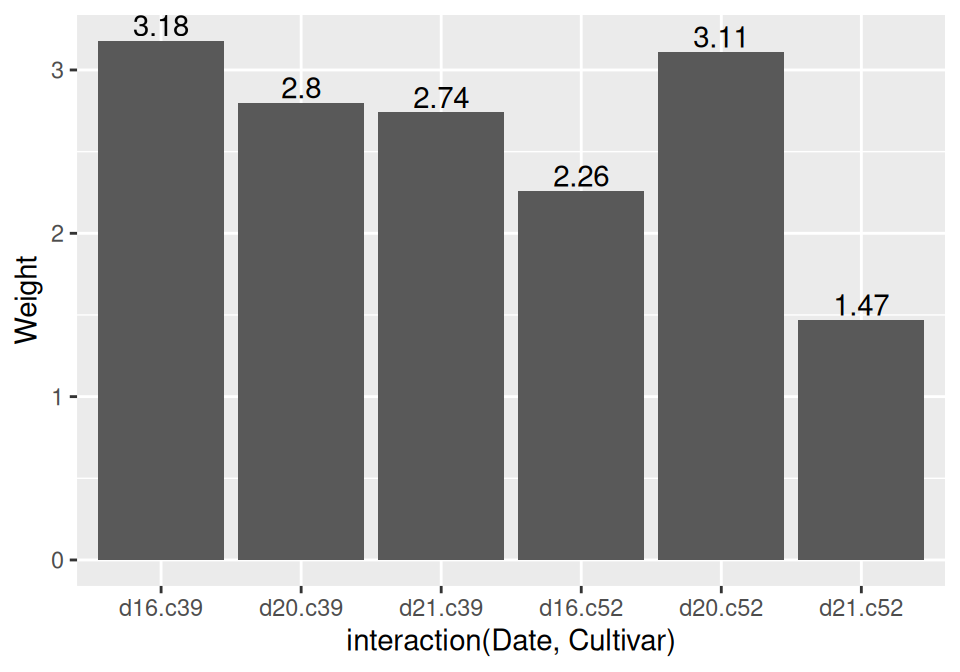Figure 3.22: Labels under the tops of bars (left); Labels above bars (right)

Notice that when the labels are placed atop the bars, they may be clipped. To remedy this, see Recipe 8.2.

Another common scenario is to add labels for a bar graph of counts instead of values. To do this, use `geom_bar()`, which adds bars whose height is proportional to the number of rows, and then use `geom_text()` with counts:

``````ggplot(mtcars, aes(x = factor(cyl))) +
geom_bar() +
geom_text(aes(label = ..count..), stat = "count", vjust = 1.5, colour = "white")``````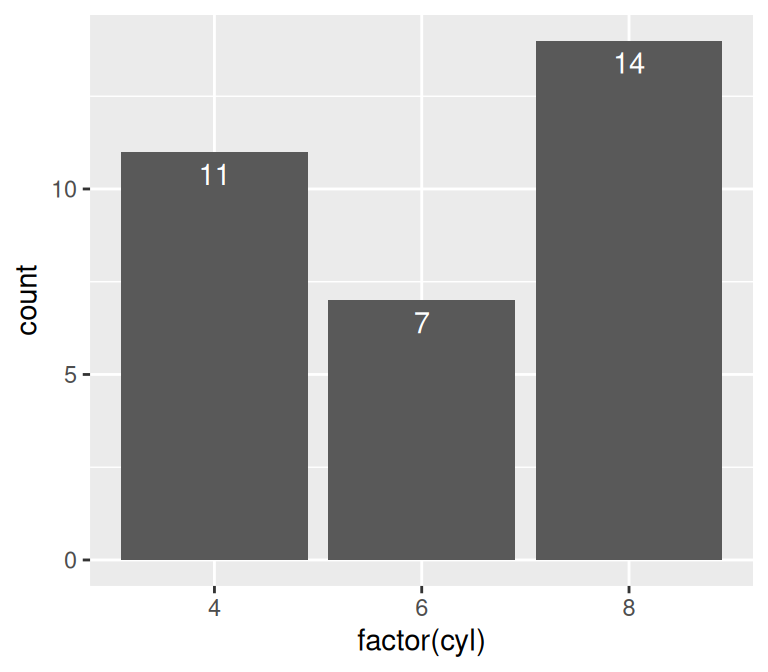Figure 3.23: Bar graph of counts with labels under the tops of bars

We needed to tell `geom_text()` to use the `"count"` statistic to compute the number of rows for each x value, and then, to use those computed counts as the labels, we told it to use the aesthetic mapping `aes(label = ..count..)`.

### 3.9.3 Discussion

In Figure 3.22, the y coordinates of the labels are centered at the top of each bar; by setting the vertical justification (`vjust`), they appear below or above the bar tops. One drawback of this is that when the label is above the top of the bar, it can go off the top of the plotting area. To fix this, you can manually set the y limits, or you can set the y positions of the text above the bars and not change the vertical justification. One drawback to changing the text’s y position is that if you want to place the text fully above or below the bar top, the value to add will depend on the y range of the data; in contrast, changing `vjust` to a different value will always move the text the same distance relative to the height of the bar:

``````# Adjust y limits to be a little higher
ggplot(cabbage_exp, aes(x = interaction(Date, Cultivar), y = Weight)) +
geom_col() +
geom_text(aes(label = Weight), vjust = -0.2) +
ylim(0, max(cabbage_exp\$Weight) * 1.05)

# Map y positions slightly above bar top - y range of plot will auto-adjust
ggplot(cabbage_exp, aes(x = interaction(Date, Cultivar), y = Weight)) +
geom_col() +
geom_text(aes(y = Weight + 0.1, label = Weight))``````

For grouped bar graphs, you also need to specify position=position_dodge() and give it a value for the dodging width. The default dodge width is 0.9. Because the bars are narrower, you might need to use size to specify a smaller font to make the labels fit. The default value of size is 5, so we’ll make it smaller by using 3 (Figure 3.24):

``````ggplot(cabbage_exp, aes(x = Date, y = Weight, fill = Cultivar)) +
geom_col(position = "dodge") +
geom_text(
aes(label = Weight),
colour = "white", size = 3,
vjust = 1.5, position = position_dodge(.9)
)``````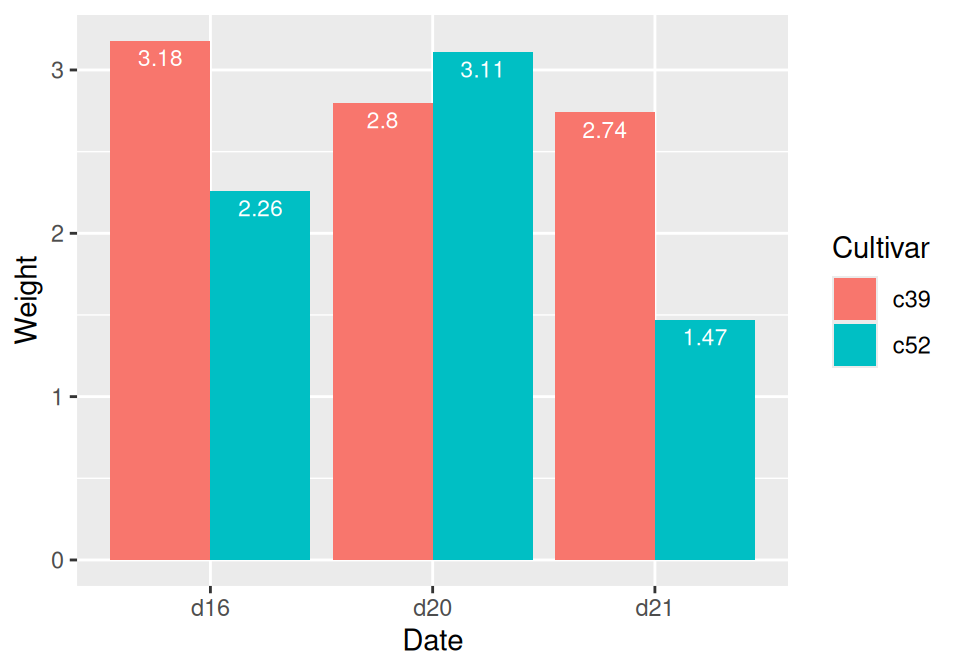Figure 3.24: Labels on grouped bars

Putting labels on stacked bar graphs requires finding the cumulative sum for each stack. To do this, first make sure the data is sorted properly – if it isn’t, the cumulative sum might be calculated in the wrong order. We’ll use the `arrange()` function from the dplyr package. Note that we have to use the `rev()` function to reverse the order of `Cultivar`:

``````library(dplyr)

# Sort by the Date and Cultivar columns
ce <- cabbage_exp %>%
arrange(Date, rev(Cultivar))``````

Once we make sure the data is sorted properly, we’ll use `group_by()` to chunk it into groups by `Date`, then calculate a cumulative sum of `Weight` within each chunk:

``````# Get the cumulative sum
ce <- ce %>%
group_by(Date) %>%
mutate(label_y = cumsum(Weight))

ce
#> # A tibble: 6 x 7
#> # Groups:   Date 
#>   Cultivar Date  Weight    sd     n     se label_y
#>   <fct>    <fct>  <dbl> <dbl> <int>  <dbl>   <dbl>
#> 1 c52      d16     2.26 0.445    10 0.141     2.26
#> 2 c39      d16     3.18 0.957    10 0.303     5.44
#> 3 c52      d20     3.11 0.791    10 0.250     3.11
#> 4 c39      d20     2.8  0.279    10 0.0882    5.91
#> 5 c52      d21     1.47 0.211    10 0.0667    1.47
#> 6 c39      d21     2.74 0.983    10 0.311     4.21

ggplot(ce, aes(x = Date, y = Weight, fill = Cultivar)) +
geom_col() +
geom_text(aes(y = label_y, label = Weight), vjust = 1.5, colour = "white")``````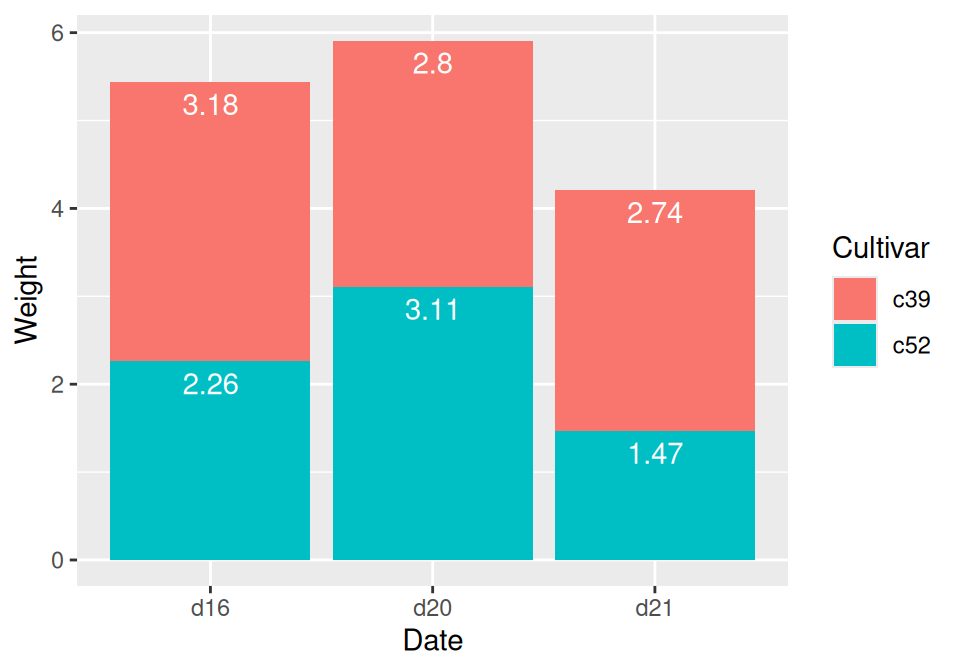Figure 3.25: Labels on stacked bars

The result is shown in Figure 3.25.

When using labels, changes to the stacking order are best done by modifying the order of levels in the factor (see Recipe 15.8) before taking the cumulative sum. The other method of changing stacking order, by specifying breaks in a scale, won’t work properly, because the order of the cumulative sum won’t be the same as the stacking order.

To put the labels in the middle of each bar (Figure 3.26), there must be an adjustment to the cumulative sum, and the y offset in `geom_bar()` can be removed:

``````ce <- cabbage_exp %>%
arrange(Date, rev(Cultivar))

# Calculate y position, placing it in the middle
ce <- ce %>%
group_by(Date) %>%
mutate(label_y = cumsum(Weight) - 0.5 * Weight)

ggplot(ce, aes(x = Date, y = Weight, fill = Cultivar)) +
geom_col() +
geom_text(aes(y = label_y, label = Weight), colour = "white")``````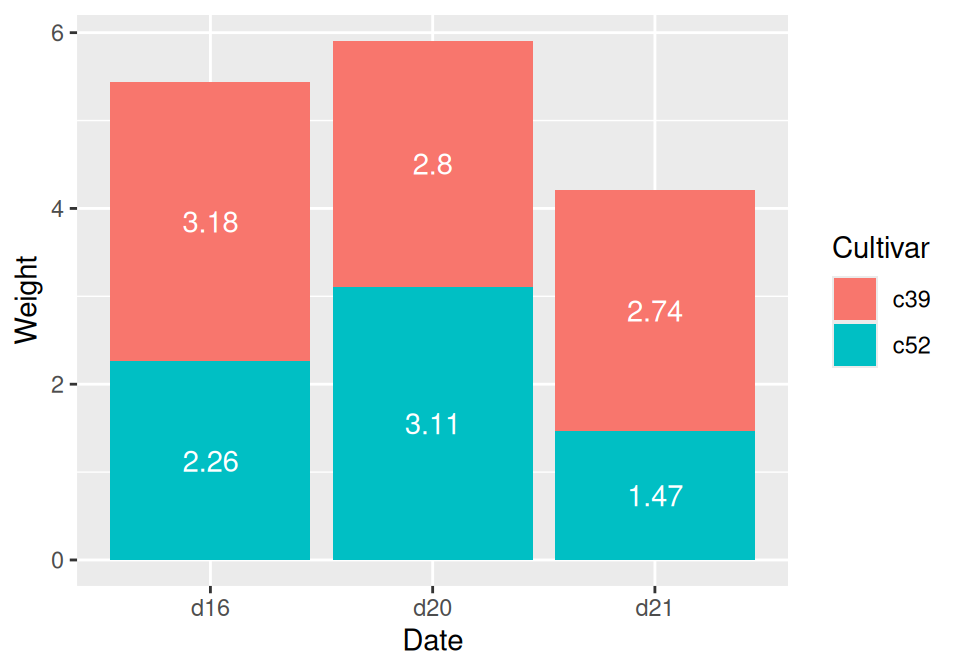Figure 3.26: Labels in the middle of stacked bars

For a more polished graph (Figure 3.27), we’ll change the colors, add labels in the middle with a smaller font using `size`, add a “kg” suffix using `paste`, and make sure there are always two digits after the decimal point by using `format()`:

``````ggplot(ce, aes(x = Date, y = Weight, fill = Cultivar)) +
geom_col(colour = "black") +
geom_text(aes(y = label_y, label = paste(format(Weight, nsmall = 2), "kg")), size = 4) +
scale_fill_brewer(palette = "Pastel1")``````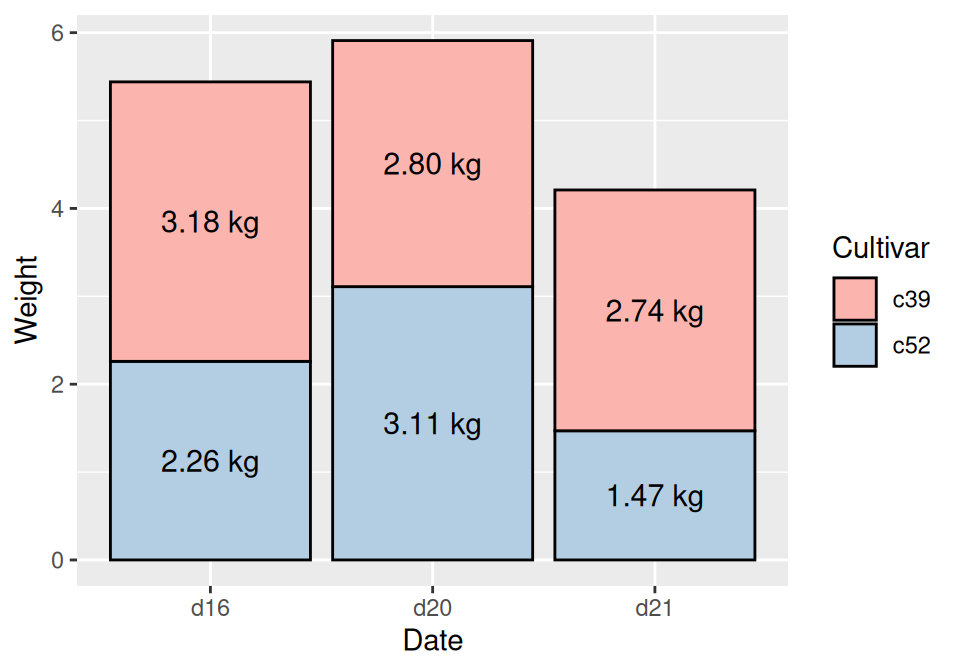Figure 3.27: Customized stacked bar graph with labels

To control the appearance of the text, see Recipe 9.2.

For more on transforming data by groups, see Recipe 15.16.

## 3.10 Making a Cleveland Dot Plot

### 3.10.1 Problem

You want to make a Cleveland dot plot.

### 3.10.2 Solution

Cleveland dot plots are an alternative to bar graphs that reduce visual clutter and can be easier to read.

The simplest way to create a dot plot (as shown in Figure 3.28) is to use `geom_point()`:

``````library(gcookbook) # Load gcookbook for the tophitters2001 data set
tophit <- tophitters2001[1:25, ] # Take the top 25 from the tophitters data set

ggplot(tophit, aes(x = avg, y = name)) +
geom_point()``````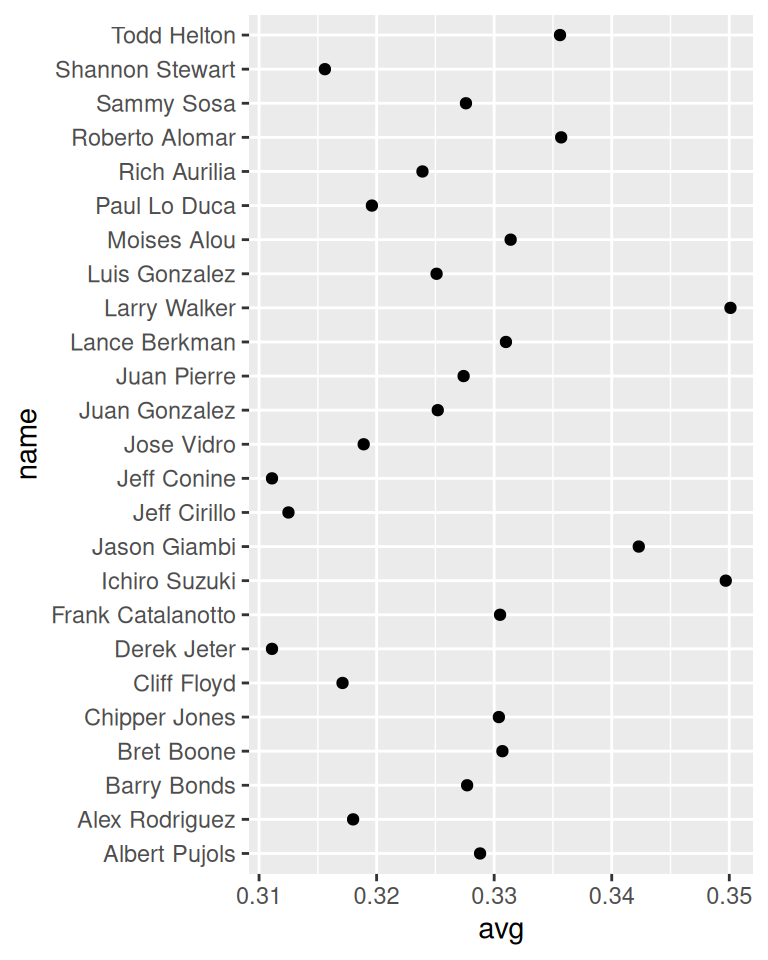Figure 3.28: Basic dot plot

### 3.10.3 Discussion

The `tophitters2001` data set contains many columns, but we’ll focus on just three of them for this example:

``````tophit[, c("name", "lg", "avg")]
#>             name lg    avg
#> 1   Larry Walker NL 0.3501
#> 2  Ichiro Suzuki AL 0.3497
#> 3   Jason Giambi AL 0.3423
#>  ...<19 more rows>...
#> 23  Jeff Cirillo NL 0.3125
#> 24   Jeff Conine AL 0.3111
#> 25   Derek Jeter AL 0.3111``````

In Figure 3.28 the names are sorted alphabetically, which isn’t very useful in this graph. Dot plots are often sorted by the value of the continuous variable on the horizontal axis.

Although the rows of `tophit` happen to be sorted by `avg`, that doesn’t mean that the items will be ordered that way in the graph. By default, the items on the given axis will be ordered however is appropriate for the data type. `name` is a character vector, so it’s ordered alphabetically. If it were a factor, it would use the order defined in the factor levels. In this case, we want `name` to be sorted by a different variable, `avg`.

To do this, we can use `reorder(name, avg)`, which takes the name column, turns it into a factor, and sorts the factor levels by `avg`. To further improve the appearance, we’ll make the vertical grid lines go away by using the theming system, and turn the horizontal grid lines into dashed lines (Figure 3.29):

``````ggplot(tophit, aes(x = avg, y = reorder(name, avg))) +
geom_point(size = 3) +  # Use a larger dot
theme_bw() +
theme(
panel.grid.major.x = element_blank(),
panel.grid.minor.x = element_blank(),
panel.grid.major.y = element_line(colour = "grey60", linetype = "dashed")
)``````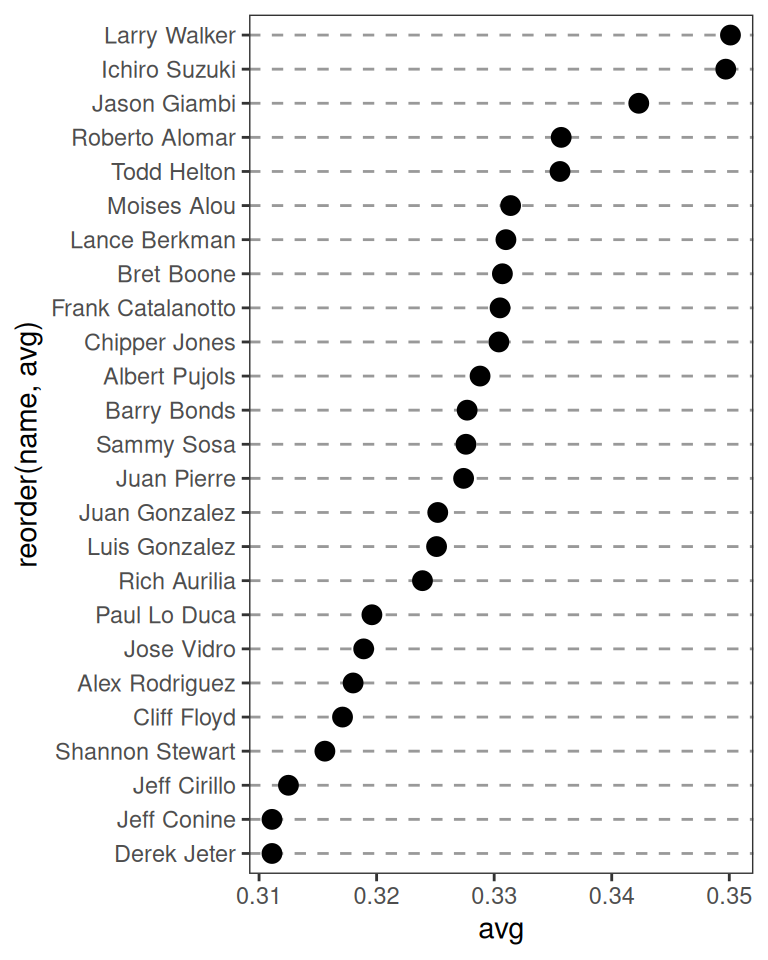Figure 3.29: Dot plot, ordered by batting average

It’s also possible to swap the axes so that the names go along the x-axis and the values go along the y-axis, as shown in Figure 3.30. We’ll also rotate the text labels by 60 degrees:

``````ggplot(tophit, aes(x = reorder(name, avg), y = avg)) +
geom_point(size = 3) +  # Use a larger dot
theme_bw() +
theme(
panel.grid.major.y = element_blank(),
panel.grid.minor.y = element_blank(),
panel.grid.major.x = element_line(colour = "grey60", linetype = "dashed"),
axis.text.x = element_text(angle = 60, hjust = 1)
)``````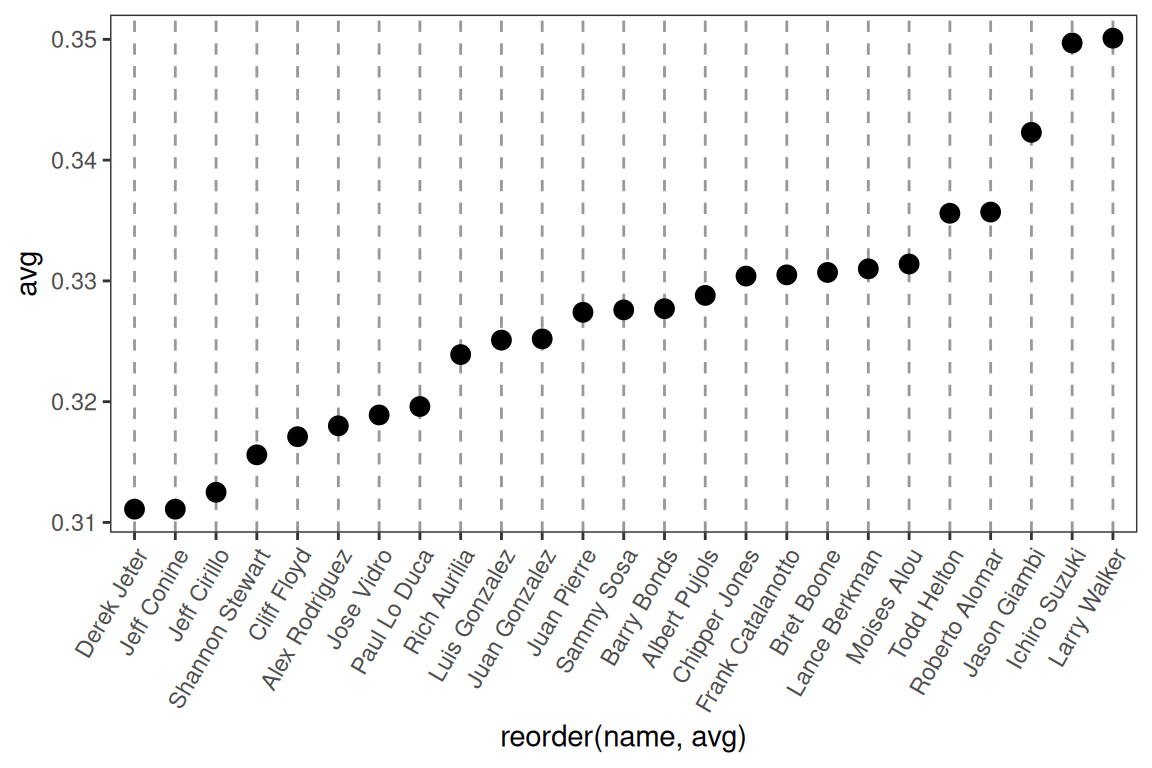Figure 3.30: Dot plot with names on x-axis and values on y-axis

It’s also sometimes desirable to group the items by another variable. In this case we’ll use the factor `lg`, which has the levels `NL` and `AL`, representing the National League and the American League. This time we want to sort first by `lg` and then by `avg`. Unfortunately, the `reorder()` function will only order factor levels by one other variable; to order the factor levels by two variables, we must do it manually:

``````# Get the names, sorted first by lg, then by avg
nameorder <- tophit\$name[order(tophit\$lg, tophit\$avg)]

# Turn name into a factor, with levels in the order of nameorder
tophit\$name <- factor(tophit\$name, levels = nameorder)``````

To make the graph (Figure 3.31), we’ll also add a mapping of `lg` to the color of the points. Instead of using grid lines that run all the way across, this time we’ll make the lines go only up to the points, by using `geom_segment()`. Note that `geom_segment()` needs values for `x`, `y`, `xend`, and `yend`:

``````ggplot(tophit, aes(x = avg, y = name)) +
geom_segment(aes(yend = name), xend = 0, colour = "grey50") +
geom_point(size = 3, aes(colour = lg)) +
scale_colour_brewer(palette = "Set1", limits = c("NL", "AL")) +
theme_bw() +
theme(
panel.grid.major.y = element_blank(),   # No horizontal grid lines
legend.position = c(1, 0.55),           # Put legend inside plot area
legend.justification = c(1, 0.5)
)``````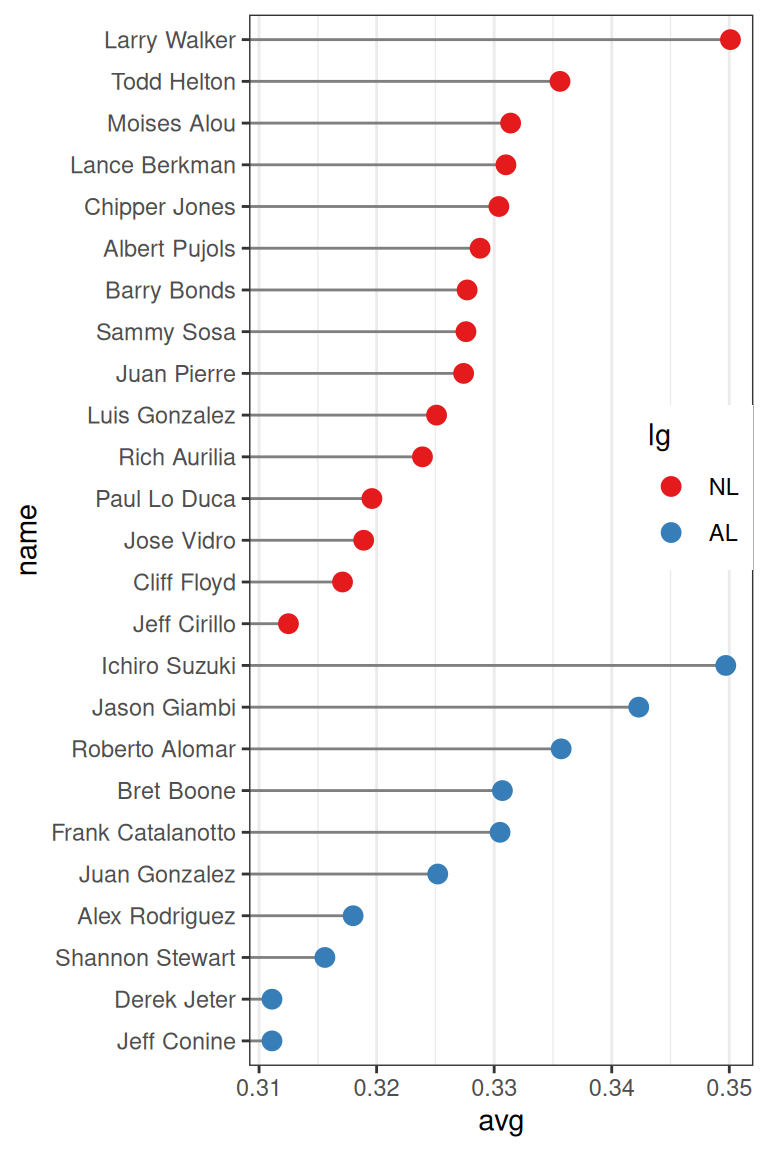Figure 3.31: Grouped by league, with lines that stop at the point

Another way to separate the two groups is to use facets, as shown in Figure 3.32. The order in which the facets are displayed is different from the sorting order in Figure 3.31; to change the display order, you must change the order of factor levels in the `lg` variable:

``````ggplot(tophit, aes(x = avg, y = name)) +
geom_segment(aes(yend = name), xend = 0, colour = "grey50") +
geom_point(size = 3, aes(colour = lg)) +
scale_colour_brewer(palette = "Set1", limits = c("NL", "AL"), guide = FALSE) +
theme_bw() +
theme(panel.grid.major.y = element_blank()) +
facet_grid(lg ~ ., scales = "free_y", space = "free_y")``````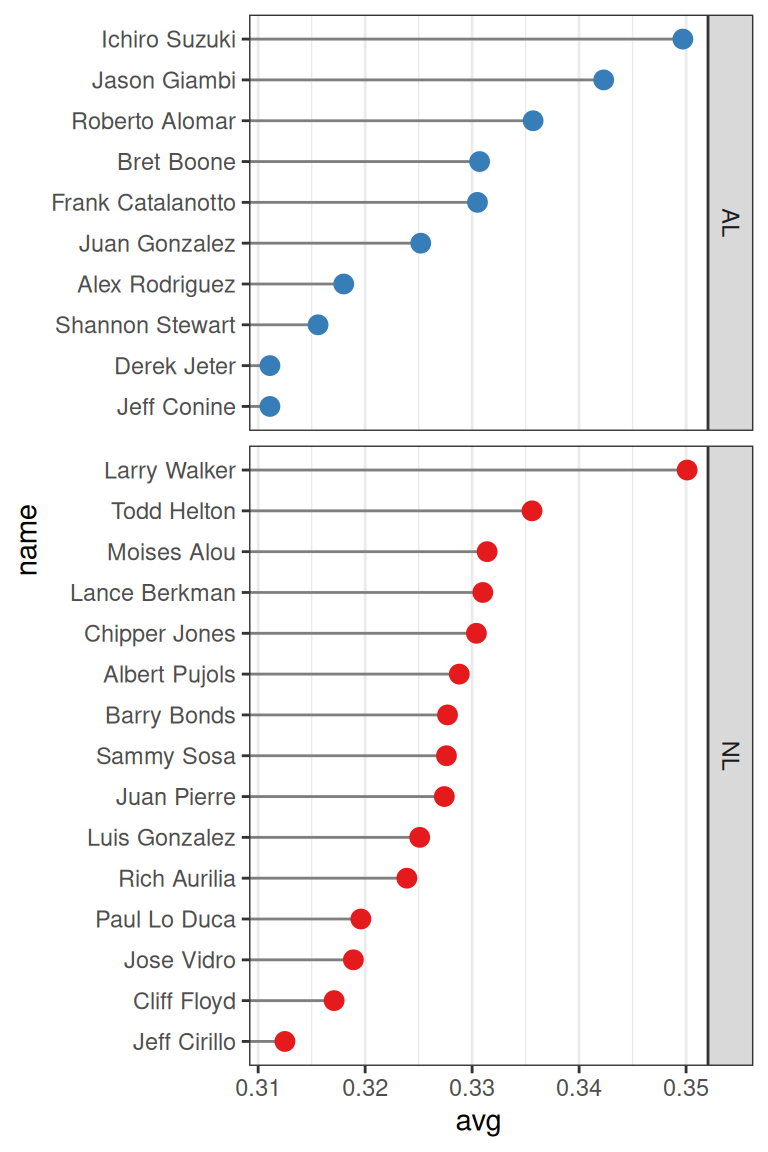Figure 3.32: Faceted by league